The Australian Journal of Mathematical Analysis and Applications

 Home News Editors Volumes RGMIA Subscriptions Authors Contact

ISSN 1449-5910

You searched for chang
Total of 351 results found in site

30: Paper Source PDF document

Paper's Title:

Hermite-Hadamard Type Inequalities for MN-Convex Functions

Author(s):

Sever S. Dragomir1,2

1Mathematics, College of Engineering & Science
Victoria University, PO Box 14428
Melbourne City, MC 8001,
Australia
E-mail: sever.dragomir@vu.edu.au

2DST-NRF Centre of Excellence in the Mathematical and Statistical Sciences,
School of Computer Science & Applied Mathematics,
University of the Witwatersrand,
Private Bag 3, Johannesburg 2050,
South Africa
URL: https://rgmia.org/dragomir

Abstract:

The present work endeavours to briefly present some of the fundamental results connected to the Hermite-Hadamard inequality for special classes of convex functions such as AG, AH, GA, GG, GH, HA, HG and HH -convex functions in which the author have been involved during the last five years. For simplicity, we call these classes of functions such as MN-convex functions, where M and N stand for any of the Arithmetic (A), Geometric (G) or Harmonic (H) weighted means of positive real numbers. The survey is intended for use by both researchers in various fields of Approximation Theory and Mathematical Inequalities, domains which have grown exponentially in the last decade, as well as by postgraduate students and scientists applying inequalities in their specific areas.

11: Paper Source PDF document

Paper's Title:

Attempts to Define a Baum--Connes Map Via Localization of Categories for Inverse Semigroups

Author(s):

Bernhard Burgstaller

Departamento de Matematica,
CEP 88.040-900 Florianopolis-SC,
Brasil.
E-mail: bernhardburgstaller@yahoo.de
URL: http://mathematik.work/bernhardburgstaller/index.html

Abstract:

An induction functor in inverse semigroup equivariant KK-theory is considered, and together with %a restriction functors certain results similar to those known from the Mackey machinery are shown. It is also verified that for any so-called E-continuous inverse semigroup its equivariant KK-theory satisfies the universal property and is a triangulated category.

8: Paper Source PDF document

Paper's Title:

Optimization and Approximation for Polyhedra in Separable Hilbert Spaces

Author(s):

Paolo d'Alessandro

Department of Mathematics,
Third University of Rome,
Italy.

E-mail: pdalex45@gmail.com

Abstract:

This paper studies infinite dimensional polyhedra, covering the case in which range spaces of operators defining inequality systems are not closed. A rangespace method of linear programming is generalized to infinite dimensions and finite dimensional methods of approximation are introduced.

7: Paper Source PDF document

Paper's Title:

Existence Results for Perturbed Fractional Differential Inclusions

Author(s):

Y.-K. Chang

Department of Mathematics,
Lanzhou Jiaotong University, Lanzhou, Gansu 730070, People's
Republic of China
lzchangyk@163.com

Abstract:

This paper is mainly concerned with the following fractional differential inclusions with boundary condition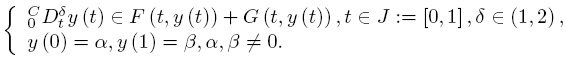A sufficient condition is established for the existence of solutions of the above problem by using a fixed point theorem for multivalued maps due to Dhage. Our result is proved under the mixed generalized Lipschitz and Carathéodory conditions.

7: Paper Source PDF document

Paper's Title:

End-Point and Transversality Conditions in the Calculus of Variations: Derivations through Direct Reasoning

Author(s):

Olivier de La Grandville

Stanford University,
Department of Management Science and Engineering,
475 Via Ortega, Stanford, CA 94305,
U. S. A.

E-mail: ola@stanford.edu

Abstract:

We offer an intuitive explanation of the end-point and transversality conditions that complement the Euler equation in the calculus of variations. Our reasoning is based upon the fact that any variation given to an optimal function must entail a zero net gain to the functional, all consequences of implied changes in its derivative being fully taken into account.

6: Paper Source PDF document

Paper's Title:

Analysis of the Flow Field in Stenosed Bifurcated Arteries Through a Mathematical Model

Author(s):

S. Chakravarty and S. Sen

Department of Mathematics, Visva-Bharati University,
Santiniketan 731235,
India
santabrata2004@yahoo.co.in

Abstract:

The present study is dealt with an appropriate mathematical model of the arotic bifurcation in the presence of constrictions using which the physiological flow field is analized. The geometry of the bifurcated arterial segment having constrictions in both the parent and its daughter arterial lumen frequently occurring in the diseased arteries causing malfunction of the cardiovascular system , is formed mathematically with the introduction of appropriate curvatures at the lateral junctions and the flow divider. The flowing blood contained in the stenosed bifurcated artery is treated to be Newtonian and the flow is considered to be two dimensional. The motion of the arterial wall and its effect on local fluid mechanics is not ruled out from the present pursuit. The flow analysis applies the time-dependent, two-dimensional incompressible nonlinear Navier-Stokes equations for Newtonian fluid. The flow field can be obtained primarily following the radial coordinate transformation and using the appropriate boundary conditions and finally adopting a suitable finite difference scheme numerically. The influences of the arterial wall distensibility and the presence of stenosis on the flow field, the flow rate and the wall shear stresses are quantified in order to indicate the susceptibility to atherosclerotic lesions and thereby to validate the applicability of the present theoretical model.

6: Paper Source PDF document

Paper's Title:

On Some Ramanujan's Schläfli Type Modular Equations

Author(s):

K. R. Vasuki

Department of Mathematics, Acharya Institute of Technology, Soldevanahalli,
Chikkabanavara (Post), Hesaragatta Main Road, Bangalore-560 090,
INDIA.
vasuki_kr@hotmail.com

Abstract:

In this paper, we give new proof of certain Ramanujan-Schläfli modular equations. We also obtain a new modular equation of degree 23.

6: Paper Source PDF document

Paper's Title:

On a Hilbert-type Inequality with the Polygamma Function

Author(s):

Bing He and Bicheng Yang
Department of Mathematics, Guangdong Education College,
Guangzhou, 510303,
China.

Department of Mathematics, Guangdong Education College,
Guangzhou, 510303,
China.

Abstract:

By applying the method of weight function and the technique of real analysis, a Hilbert-type inequality with a best constant factor is established, where the best constant factor is made of the polygamma function. Furthermore, the inverse form is given.

6: Paper Source PDF document

Paper's Title:

Optimal Control Analysis of HIV/AIDS Epidemic Model with an Antiretroviral Treatment

Author(s):

U. Habibah and R. A. Sari

Mathematics Department and Reseach Group of Biomathematics,
Faculty of Mathematics and Natural Science,
Brawijaya University, Jl. Veteran Malang 65145,
Indonesia.
E-mail: ummu_habibah@ub.ac.id

Abstract:

A mathematical model of HIV/AIDS is governed by a system of ordinary differential equations in the presence of an antiretroviral treatment (ARV). The theory of optimal control is applied to an epidemic model of HIV/AIDS which an ARV is used as a control strategy in order to prevent the spread of HIV/AIDS. The optimality system is derived by applying the Pontryagin's Minimum Principle. We analyze the boundedness and positivity of solutions, and an existence of the optimal control. Numerical simulations are conducted to obtain numerical solution of the optimally system.

6: Paper Source PDF document

Paper's Title:

Coexisting Attractors and Bubbling Route to Chaos in Modified Coupled Duffing Oscillators

Author(s):

B. Deruni1, A. S. Hacinliyan1,2, E. Kandiran3, A. C. Keles2, S. Kaouache4, M.-S. Abdelouahab4, N.-E. Hamri4

1Department of Physics,
University of Yeditepe,
Turkey.

2Department of Information Systems and Technologies,
University of Yeditepe,
Turkey

3Department of Software Development,
University of Yeditepe,
Turkey.

4Laboratory of Mathematics and their interactions,
University Center of Abdelhafid Boussouf,
Mila 43000,
Algeria.

E-mail: berc890@gmail.com
ahacinliyan@yeditepe.edu.tr
engin.kandiran@yeditepe.edu.tr
cihan.keles@yeditepe.edu.tr
s.kaouache@centr-univ-mila.dz
medsalah3@yahoo.fr
n.hamri@centre-univ-mila.dz

Abstract:

In this article dynamical behavior of coupled Duffing oscillators is analyzed under a small modification. The oscillators have cubic damping instead of linear one. Although single duffing oscillator has complex dynamics, coupled duffing systems possess a much more complex structure. The dynamical behavior of the system is investigated both numerically and analytically. Numerical results indicate that the system has double scroll attractor with suitable parameter values. On the other hand, bifurcation diagrams illustrate rich behavior of the system, and it is seen that, system enters into chaos with different routes. Beside classical bifurcations, bubbling route to chaos is observed for suitable parameter settings. On the other hand, Multistability of the system is indicated with the coexisting attractors, such that under same parameter setting the system shows different periodic and chaotic attractors. Moreover, chaotic synchronization of coupled oscillators is illustrated in final section.

5: Paper Source PDF document

Paper's Title:

The Convergence of Modified Mann-Ishikawa Iterations when Applied to an Asymptotically Pseudocontractive Map

Author(s):

S. Soltuz

Departamento de Matematicas, Universidad de Los Andes, Carrera 1
No. 18A-10, Bogota,
Colombia
and
``T. Popoviciu" Institute of Numerical Analysis
Cluj-Napoca,
Romania
smsoltuz@gmail.com
URL:http://www.uniandes.edu.co/

Abstract:

We prove that under minimal conditions the modified Mann and Ishikawa iterations converge when dealing with an asymptotically pseudocontractive map. We give an affirmative answer to the open question from C.E. Chidume and H. Zegeye, Approximate fixed point sequences and convergence theorems for asymptotically pseudocontractive mappings, J. Math. Anal. Appl., 278 (2003), 354--366.

5: Paper Source PDF document

Paper's Title:

Long Correlations Applied to the Study of Agricultural Indices in Comparison with the S&P500 index

Author(s):

M. C. Mariani, J. Libbin, M.P. Beccar Varela,
V. Kumar Mani, C. Erickson, D.J. Valles Rosales

Department of Mathematical Sciences,
Science Hall 236, New Mexico State University,
Las Cruces, NM 88003-8001,
USA.
mmariani@nmsu.edu

Abstract:

Long-time correlations in agricultural indices are studied and their behavior is compared to the well-established S&P500 index. Hurst exponent and Detrended Fluctuation Analysis (DFA) techniques are used in this analysis. We detected long-correlations in the agricultural indices and briefly discussed some features specific in comparison to the S&P500 index.

5: Paper Source PDF document

Paper's Title:

A Note On The Global Behavior Of A Nonlinear System of Difference Equations

Author(s):

Norman H. Josephy, Mihaela Predescu and Samuel W. Woolford

Department of Mathematical Sciences,
Bentley University,
Waltham, MA 02452,
U.S.A.

mpredescu@bentley.edu
njosephy@bentley.edu
swoolford@bentley.edu

Abstract:

This paper deals with the global asymptotic stability character of solutions of a discrete time deterministic model proposed by Wikan and Eide in Bulletin of Mathematical Biology, 66, 2004, 1685-1704. A stochastic extension of this model is proposed and discussed. Computer simulations suggest that the dynamics of the stochastic model includes a mixture of the dynamics observed in the deterministic model.

5: Paper Source PDF document

Paper's Title:

Stability Problems for Generalized Additive Mappings and Euler-Lagrange Type Mappings

Author(s):

M. Todoroki, K. Kumahara, T. Miura and S.-E. Takahasi

The Open University of Japan,
Chiba, 261-8586,
Japan
tomamiyu3232@sky.sannet.ne.jp
kumahara@ouj.ac.jp

Yamagata University,
Yonezawa 992-8510,
Japan
miura@yz.yamagata-u.ac.jp

Toho University, Yamagata University,
Chiba, 273-0866,
Japan
sin_ei1@yahoo.co.jp

Abstract:

We introduce a generalized additivity of a mapping between Banach spaces and establish the Ulam type stability problem for a generalized additive mapping. The obtained results are somewhat different from the Ulam type stability result of Euler-Lagrange type mappings obtained by H. -M. Kim, K. -W. Jun and J. M. Rassias.

5: Paper Source PDF document

Paper's Title:

A One-Line Derivation of the Euler and Ostrogradski Equations

Author(s):

Olivier de La Grandville

Stanford University,
Department of Management Science and Engineering,
Stanford, CA 94305,
U. S. A

Abstract:

At the very heart of major results of classical physics, the Euler and Ostrogradski equations have apparently no intuitive interpretation. In this paper we show that this is not so. Relying on Euler's initial geometric approach, we show that they can be obtained through a direct reasoning that does not imply any calculation. The intuitive approach we suggest offers two benefits: it gives immediate significance to these fundamental second-order non-linear differential equations; and second, it allows to obtain a property of the calculus of variations that does not seem to have been uncovered until now: the Euler and Ostrogradski equations can be derived not necessarily by giving a variation to the optimal function -- as is always done; one could equally well start by giving a variation to their derivative(s).

5: Paper Source PDF document

Paper's Title:

Ostrowski Type Inequalities for Lebesgue Integral: a Survey of Recent Results

Author(s):

Sever S. Dragomir1,2

1Mathematics, School of Engineering & Science
Victoria University, PO Box 14428
Melbourne City, MC 8001,
Australia
E-mail: sever.dragomir@vu.edu.au

2DST-NRF Centre of Excellence in the Mathematical and Statistical Sciences,
School of Computer Science & Applied Mathematics,
University of the Witwatersrand,
Private Bag 3, Johannesburg 2050,
South Africa
URL: http://rgmia.org/dragomir

Abstract:

The main aim of this survey is to present recent results concerning Ostrowski type inequalities for the Lebesgue integral of various classes of complex and real-valued functions. The survey is intended for use by both researchers in various fields of Classical and Modern Analysis and Mathematical Inequalities and their Applications, domains which have grown exponentially in the last decade, as well as by postgraduate students and scientists applying inequalities in their specific areas.

5: Paper Source PDF document

Paper's Title:

Action of Differential Operators On Chirpsconstruct On L

Author(s):

Taoufik El Bouayachi and Naji Yebari

Laboratoire de Mathematiques et applications,
Faculty of sciences and techniques, Tangier,
Morocco.
E-mail: figo407@gmail.com, yebarinaji@gmail.com

Abstract:

We will study in this work the action of differential operators on L chirps and we will give a new definition of logarithmic chirp. Finally we will study the action of singular integral operators on chirps by wavelet characterization and Kernel method.

5: Paper Source PDF document

Paper's Title:

Lie Group Theoretic Approach of One-Dimensional Black-Scholes Equation

Author(s):

P. L. Zondi and M. B. Matadi

Department of Mathematical sciences,
Faculty of Sciences & Agriculture, University of Zululand,
P Bag X1001, Kwa-Dlangezwa 3886,
South Africa.
zondip@unizulu.ac.za

Abstract:

This study discusses the Lie Symmetry Analysis of Black-Scholes equation via a modified local one-parameter transformations. It can be argued that the transformation of the Black-Scholes equation is firstly obtained by means of riskless rate. Thereafter, the corresponding determining equations to the reduced equation are found. Furthermore, new symmetries of the Black-Scholes equation are constructed and lead to invariant solutions.

4: Paper Source PDF document

Paper's Title:

New Proofs of the Grüss Inequality

Author(s):

A.Mc.D. Mercer and Peter R. Mercer

Dept. Mathematics and Statistics,
University of Guelph, Guelph, Ontario,
amercer@reach.net

Dept. Mathematics,
S.U.N.Y. College at Buffalo, Buffalo, NY,
U.S.A.
mercerpr@math.buffalostate.edu

Abstract:

We present new proofs of the Grüss inequality in its original form and in its linear functional form.

4: Paper Source PDF document

Paper's Title:

Generalizing Polyhedra to Infinite Dimension

Author(s):

Paolo d'Alessandro

Department of Mathematics, Third University of Rome,
Lgo S.L. Murialdo 1, 00146 Rome, Italy.

dalex@mat.uniroma3.it.

URL: http://www.mat.uniroma3.it/users/dalex/dalex.html.

Abstract:

This paper generalizes polyhedra to infinite dimensional Hilbert spaces as countable intersections of closed semispaces. Highlights are the structure theory that shows that a polyhedron is the sum of compact set (in a suitable topology) plus a closed pointed cone plus a closed subspace, giving the internal representation of polyhedra. In the final part the dual range space technique is extended to the solution of infinite dimensional LP problems.

4: Paper Source PDF document

Paper's Title:

On a Problem on Periodic Functions

Author(s):

Mathematics Department, Faculty of Science,
Jerash Private University, Jerash,
Jordan.

Abstract:

Given a continuous periodic real function f with n translates  f1 ,..., fn , where fi(x)=f(x+ai), i=1,...,n. We solve a problem by Erdos and Chang and show that there are rational numbers r,s such that f(r)≥ fi(r), f(s)≤ fi(s), i=1,...,n. No restrictions on the constants or any further restriction on the function f are necessary as was imposed earlier.

4: Paper Source PDF document

Paper's Title:

A Comparison Between Two Different Stochastic Epidemic Models with Respect to the Entropy

Author(s):

Department of Mathematics,
University of Sussex,
Brighton BN1 9QH,
UK.
E-mail: f.fatehi@sussex.ac.uk
URL: http://www.sussex.ac.uk/profiles/361251

Department of Pure Mathematics, Faculty of Mathematics and Computer,
Shahid Bahonar University of Kerman,
Kerman 76169-14111,
Iran.

Abstract:

In this paper at first a brief history of mathematical models is presented with the aim to clarify the reliability of stochastic models over deterministic models. Next, the necessary background about random variables and stochastic processes, especially Markov chains and the entropy are introduced. After that, entropy of SIR stochastic models is computed and it is proven that an epidemic will disappear after a long time. Entropy of a stochastic mathematical model determines the average uncertainty about the outcome of that random experiment. At the end, we introduce a chain binomial epidemic model and compute its entropy, which is then compared with the DTMC SIR epidemic model to show which one is nearer to reality.

4: Paper Source PDF document

Paper's Title:

An Existence of the Solution to Neutral Stochastic Functional Differential Equations Under the Holder Condition

Author(s):

Young-Ho Kim

Department of Mathematics,
Changwon National University,
Changwon, Gyeongsangnam-do 51140,
Korea.

E-mail: iyhkim@changwon.ac.kr

Abstract:

In this paper, we show the existence and uniqueness of solution of the neutral stochastic functional differential equations under weakened H\"{o}lder condition, a weakened linear growth condition, and a contractive condition. Furthermore, in order to obtain the existence of a solution to the equation we used the Picard sequence.

3: Paper Source PDF document

Paper's Title:

Viability Theory And Differential Lanchester Type Models For Combat.
Differential Systems.

Author(s):

G. Isac and A. Gosselin

Department Of Mathematics, Royal Military College Of Canada,
P.O. Box 17000, S
tn Forces, Kingston, Ontario, Canada K7k 7b4

URL
:

URL
:

Abstract:

In 1914, F.W. Lanchester proposed several mathematical models based on differential equations to describe combat situations . Since then, his work has been extensively modified to represent a variety of competitions including entire wars. Differential Lanchester type models have been studied from many angles by many authors in hundreds of papers and reports. Lanchester type models are used in the planning of optimal strategies, supply and tactics. In this paper, we will show how these models can be studied from a viability theory stand point. We will introduce the notion of winning cone and show that it is a viable cone for these models. In the last part of our paper we will use the viability theory of differential equations to study Lanchester type models from the optimal theory point of view.

3: Paper Source PDF document

Paper's Title:

Boundary Value Problems for Fractional Diffusion-Wave equation

Author(s):

Varsha Daftardar-Gejji and Hossein Jafari

Department of Mathematics, University of Pune,
Ganeshkhind, Pune - 411007,
INDIA.
vsgejji@math.unipune.ernet.in
jafari_h@math.com

Abstract:

Non homogeneous fractional diffusion-wave equation has been solved under linear/nonlinear boundary conditions. As the order of time derivative changes from 0 to 2, the process changes from slow diffusion to classical diffusion to mixed diffusion-wave behaviour.
Numerical examples presented here confirm this inference. Orthogonality of eigenfunctions in case of fractional Stürm-Liouville problem has been established

3: Paper Source PDF document

Paper's Title:

Numerical Studies on Dynamical Systems Method for Solving Ill-posed Problems with Noise

Author(s):

N. H. Sweilam and A. M. Nagy

Mathematics Department, Faculty of Science,
Cairo University,
Giza, Egypt.
n_sweilam@yahoo.com

Mathematics Department, Faculty of Science,
Benha University,
Benha, Egypt.
abdelhameed_nagy@yahoo.com

Abstract:

In this paper, we apply the dynamical systems method proposed by A. G. RAMM, and the the variational regularization method to obtain numerical solution to some ill-posed problems with noise. The results obtained are compared to exact solutions. It is found that the dynamical systems method is preferable because it is easier to apply, highly stable, robust, and it always converges to the solution even for large size models.

3: Paper Source PDF document

Paper's Title:

Existence of Bounded Solutions for a Class of Strongly Nonlinear Elliptic Equations in Orlicz-Sobolev Spaces

Author(s):

Abdelmoujib Benkirane and Ahmed Youssfi

Department of Mathematics and Informatics, Faculty of Sciences Dhar El Mahraz
University Sidi Mohammed Ben Abdallah
PB 1796 Fez-Atlas, Fez
Morocco
a.benkirane@menara.ma
ahmed.youssfi@caramail.com

Abstract:

We prove, in the setting of Orlicz-Sobolev spaces, the existence of bounded solutions for some strongly nonlinear elliptic equations with operator of the principal part having degenerate coercivity and lower order terms not satisfying the sign condition. The data have a suitable summability and no Δ2-condition is needed for the considered N-functions.

3: Paper Source PDF document

Paper's Title:

Linearly Transformable Minimal Surfaces

Author(s):

Harold R. Parks and Walter B. Woods

Department of Mathematics, Oregon State University,
Corvallis, Oregon 97331--4605,
USA
parks@math.oregonstate.edu
URL
: http://www.math.oregonstate.edu/people/view/parks/

Abstract:

We give a complete description of a nonplanar minimal surface in R3 with the surprising property that the surface remains minimal after mapping by a linear transformation that dilates by three distinct factors in three orthogonal directions. The surface is defined in closed form using Jacobi elliptic functions.

3: Paper Source PDF document

Paper's Title:

Ergodic Solenoidal Homology II: Density of Ergodic Solenoids

Author(s):

Vicente Muńoz and Ricardo Pérez Marco

Instituto de Ciencias Matemáticas CSIC-UAM-UC3M-UCM,
Spain
and
Plaza de Ciencias 3, 28040 Madrid,
Spain

CNRS, LAGA UMR 7539, Universit
é Paris XIII,
99 Avenue J.-B. Cl\'ement, 93430-Villetaneuse,
France

vicente.munoz@imaff.cfmac.csic.es
ricardo@math.univ-paris13.fr

Abstract:

A measured solenoid is a laminated space endowed with a tranversal measure invariant by holonomy. A measured solenoid immersed in a smooth manifold produces a closed current (known as a generalized Ruelle-Sullivan current). Uniquely ergodic solenoids are those for which there is a unique (up to scalars) transversal measure. It is known that for any smooth manifold, any real homology class is represented by a uniquely ergodic solenoid. In this paper, we prove that the currents associated to uniquely ergodic solenoids are dense in the space of closed currents, therefore proving the abundance of such objects.

3: Paper Source PDF document

Paper's Title:

Nontrivial Solutions of Singular Superlinear Three-point Boundary Value Problems at Resonance

Author(s):

Feng Wang, Fang Zhang

School of Mathematics and Physics,
Changzou University,
Changzhou, 213164,
China.
fengwang188@163.com

Abstract:

The singular superlinear second order three-point boundary value problems at resonance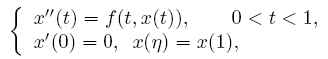are considered under some conditions concerning the first eigenvalues corresponding to the relevant linear operators, where n ∈ (0,1) is a constant, f is allowed to be singular at both t=0 and t=1. The existence results of nontrivial solutions are given by means of the topological degree theory.

3: Paper Source PDF document

Paper's Title:

Hardy Type Inequalities via Convexity - The Journey so Far

Author(s):

James A. Oguntuase and Lars-Erik Persson

Department of Mathematics, University of Agriculture,
P. M. B. 2240, Abeokuta, Nigeria.

Department of Mathematics, Luleĺ University of Technology,
SE-971 87, Luleĺ , Sweden.

oguntuase@yahoo.com, larserik@sm.luth.se .

Abstract:

It is nowadays well-known that Hardy's inequality (like many other inequalities) follows directly from Jensen's inequality. Most of the development of Hardy type inequalities has not used this simple fact, which obviously was unknown by Hardy himself and many others. Here we report on some results obtained in this way mostly after 2002 by mainly using this fundamental idea.

3: Paper Source PDF document

Paper's Title:

On Some Relations Among the Solutions of the Linear Volterra Integral Equations

Author(s):

Ismet Ozdemir and Faruk Temizer

Inönü Üniversitesi Eğitim Fakültesi,
44280-Malatya,
Turkey

ismet.ozdemir@inonu.edu.tr

omer.temizer@inonu.edu.tr

Abstract:

The sufficient conditions for y1(x)≤ y2(x) were given in  such that ym(x)=fm(x)+∫ax Km(x, t)ym(t)dt,(m=1,2) and x∈ [a, b]. Some properties such as positivity, boundedness and monotonicity of the solution of the linear Volterra integral equation of the form f(t)=1-∫0tK(t-τ)f(τ)dτ=1-K*f, (0≤ t<∞) were obtained, without solving this equation, in [3,4,5,6]. Also, the boundaries for functions f', f'',..., f(n),(n ∈ N) defined on the infinite interval [0, ∞) were found in [7,8].

In this work, for the given equation f(t)=1-K* f and n≥ 2, it is derived that there exist the functions  L2, L3,..., Ln which can be obtained by means of K and some inequalities among the functions f, h2, h3,..., hi for i=2, 3,...., n are satisfied on the infinite interval [0, ∞), where hi is the solution of the equation hi(t)=1-Li* hi and n is a natural number.

3: Paper Source PDF document

Paper's Title:

Some Properties of the Marshall-Olkin and Generalized Cuadras-Augé Families of Copulas

Author(s):

Edward Dobrowolski and Pranesh Kumar

Department of Mathematics and Statistics
University of Northern British Columbia
Prince George, BC,

E-mail: Pranesh.Kumar@unbc.ca

Abstract:

We investigate some properties of the families of two parameter Marshall-Olkin and Generalized Cuadras-Augé copulas. Some new results are proved for copula parameters, dependence measure and mutual information. A numerical application is discussed.

3: Paper Source PDF document

Paper's Title:

Presentation a mathematical model for bone metastases control by using tamoxifen

Author(s):

Maryam Nikbakht, Alireza Fakharzadeh Jahromi and Aghileh Heydari

Department of Mathematics,
Payame Noor University,
P.O.Box 19395-3697, Tehran,
Iran.
.E-mail: maryam_nikbakht@pnu.ac.ir

Department of Mathematics,
Faculty of Basic Science,
Shiraz University of Technology.

Department of Mathematics,
Payame Noor University,
P.O.Box 19395-3697, Tehran,
Iran.
E-mail: a-heidari@pnu.ac.ir

Abstract:

Bone is a common site for metastases (secondary tumor) because of breast and prostate cancer. According to our evaluations the mathematical aspect of the effect of drug in bone metastases has not been studied yet. Hence, this paper suggested a new mathematical model for bone metastases control by using tamoxifen. The proposed model is a system of nonlinear partial differential equations. In this paper our purpose is to present a control model for bone metastases. At end by some numerical simulations, the proposed model is examined by using physician.

3: Paper Source PDF document

Paper's Title:

Introducing the Dorfmanian: A Powerful Tool for the Calculus Of Variations

Author(s):

Olivier de La Grandville

Department of Management Science and Engineering,
Stanford University,
475 Via Ortega, Stanford, CA 94305,
U. S. A.

E-mail: odelagrandville@gmail.com

Abstract:

We show how a modified Hamiltonian proposed by Robert Dorfman  to give intuitive sense to the Pontryagin maximum principle can be extended to easily obtain all high-order equations of the calculus of variations. This new concept is particularly efficient to determine the differential equations leading to the extremals of functionals defined by n-uple integrals, while a traditional approach would require -- in some cases repeatedly -- an extension of Green's theorem to n-space.
Our paper is dedicated to the memory of Robert Dorfman (1916 - 2002).

3: Paper Source PDF document

Paper's Title:

A Method of the Study of the Cauchy Problem for~a~Singularly Perturbed Linear Inhomogeneous Differential Equation

Author(s):

E. E. Bukzhalev and A. V. Ovchinnikov

Faculty of Physics,
Moscow State University,
1 Leninskie Gory,
Moscow, 119991,
Russia
E-mail: bukzhalev@mail.ru

Russian Institute for Scientific and Technical Information of the Russian Academy of Sciences,
20 Usievicha St., Moscow, 125190,
Russia
E-mail: ovchinnikov@viniti.ru

Abstract:

We construct a sequence that converges to a solution of the Cauchy problem for a singularly perturbed linear inhomogeneous differential equation of an arbitrary order. This sequence is also an asymptotic sequence in the following sense: the deviation (in the norm of the space of continuous functions) of its nth element from the solution of the problem is proportional to the (n+1)th power of the parameter of perturbation. This sequence can be used for justification of asymptotics obtained by the method of boundary functions.

3: Paper Source PDF document

Paper's Title:

Inequalities for Functions of Selfadjoint Operators on Hilbert Spaces:
a Survey of Recent Results

Author(s):

Sever S. Dragomir1,2

1Mathematics, College of Engineering & Science
Victoria University, PO Box 14428
Melbourne City, MC 8001,
Australia
E-mail: sever.dragomir@vu.edu.au

2DST-NRF Centre of Excellence in the Mathematical and Statistical Sciences,
School of Computer Science & Applied Mathematics,
University of the Witwatersrand,
Private Bag 3, Johannesburg 2050,
South Africa
URL: https://rgmia.org/dragomir

Abstract:

The main aim of this survey is to present recent results concerning inequalities for continuous functions of selfadjoint operators on complex Hilbert spaces. It is intended for use by both researchers in various fields of Linear Operator Theory and Mathematical Inequalities, domains which have grown exponentially in the last decade, as well as by postgraduate students and scientists applying inequalities in their specific areas.

3: Paper Source PDF document

Paper's Title:

Generalised Models for Torsional Spine Reconnection

Author(s):

Ali Khalaf Hussain Al-Hachami

Department of Mathematics,
College of Education For Pure Sciences,
Wasit University,
Iraq.
E-mail: alhachamia@uowasit.edu.iq

Abstract:

Three-dimensional (3D) null points are available in wealth in the solar corona, and the equivalent is probably going to be valid in other astrophysical situations. On-going outcomes from sun oriented perceptions and from reproductions propose that reconnection at such 3D nulls may assume a significant job in the coronal dynamics. The properties of the torsional spine method of magnetic reconnection at 3D nulls are researched. Kinematic model are created, which incorporate the term ηJ that is spatially localised around the null, stretching out along the spine of the null. The null point is to research the impact of shifting the level of asymmetry of the null point magnetic field on the subsequent reconnection process where past examinations constantly considered a non-nonexclusive radially symmetric null. Specifically we analyse the rate of reconnection of magnetic flux at the spine of null point. Logical arrangements are determined for the enduring kinematic equation, and contrasted and the after effects of torsional spine reconnection models when the current is restricted in which the Maxwell conditions are illuminated. The geometry of the current layers inside which torsional spine reconnection happen is autonomous on the symmetry of the magnetic field. Torsional spine reconnection happens in a thin cylinder around the spine, with circular cross-segment when the fan eigenvalues are extraordinary. The short axis of the circle being along the solid field bearing. Just as it was discovered that the fundamental structure of the method of attractive reconnection considered is unaffected by changing the magnetic field symmetry, that is, the plasma flow is discovered rotational around the spine of null point. The spatiotemporal pinnacle current, and the pinnacle reconnection rate achieved, are found not to rely upon the level of asymmetry.

3: Paper Source PDF document

Paper's Title:

Accuracy of Implicit DIMSIMs with Extrapolation

Author(s):

A. J. Kadhim, A. Gorgey, N. Arbin and N. Razali

Mathematics Department, Faculty of Science and Mathematics,
Sultan Idris Education University,
35900 Tanjong Malim, Perak,
Malaysia
E-mail: annie_gorgey@fsmt.upsi.edu.my

Unit of Fundamental Engineering Studies,
Faculty of Engineering, Built Environment,
The National University of Malaysia,
43600 Bangi,
Malaysia.

Abstract:

The main aim of this article is to present recent results concerning diagonal implicit multistage integration methods (DIMSIMs) with extrapolation in solving stiff problems. Implicit methods with extrapolation have been proven to be very useful in solving problems with stiff components. There are many articles written on extrapolation of Runge-Kutta methods however fewer articles on extrapolation were written for general linear methods. Passive extrapolation is more stable than active extrapolation as proven in many literature when solving stiff problems by the Runge-Kutta methods. This article takes the first step by investigating the performance of passive extrapolation for DIMSIMs type-2 methods. In the variable stepsize and order codes, order-2 and order-3 DIMSIMs with extrapolation are investigated for Van der Pol and HIRES problems. Comparisons are made with ode23 solver and the numerical experiments showed that implicit DIMSIMs with extrapolation has greater accuracy than the method itself without extrapolation and ode23.

3: Paper Source PDF document

Paper's Title:

The Effect of Harvesting Activities on Prey-Predator Fishery Model with Holling type II in Toxicant Aquatic Ecosystem

Author(s):

Moh Nurul Huda, Fidia Deny Tisna Amijaya, Ika Purnamasari

Department of Mathematics, Faculty of Mathematics and Natural Science,
Mulawarman University,
Samarinda, East Kalimantan,75123
Indonesia.
E-mail: muh.nurulhuda@fmipa.unmul.ac.id
ika.purnamasari@fmipa.unmul.ac.id

Abstract:

This paper discussed prey-predator fishery models, in particular by analysing the effects of toxic substances on aquatic ecosystems. It is assumed in this model, that the prey population is plankton and the predator population is fish.\ Interaction between the two populations uses the Holling type II function. The existence of local and global critical points of the system are shown and their stability properties are analysed. Furthermore, Bionomic equilibrium and optimal control of harvesting are discussed. Finally, numerical simulations have been carried out to show in the interpretation of results.

2: Paper Source PDF document

Paper's Title:

On an Extension of Hilbert’s Integral Inequality with Some Parameters

Author(s):

Bicheng Yang

Department of Mathematics, Guangdong Education College, Guangzhou, Guangdong 510303, People’s Republic of China.
URL
:

Abstract:

In this paper, by introducing some parameters and estimating the weight function, we give an extension of Hilbert’s integral inequality with a best constant factor. As applications, we consider the equivalent form and some particular results.

2: Paper Source PDF document

Paper's Title:

Positive Solution For Discrete Three-Point Boundary Value Problems

Author(s):

Wing-Sum Cheung And Jingli Ren

Department of Mathematics,
The University of Hong Kong,
Pokfulam, Hong Kong
wscheung@hku.hk

Institute of Systems Science,
Beijing 100080, P.R. China
renjl@mx.amss.ac.cn

Abstract:

This paper is concerned with the existence of positive solution to the discrete three-point boundary value problem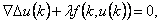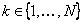,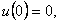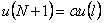where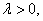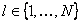, and f is allowed to change sign. By constructing available operators, we shall apply the method of lower solution and the method of topology degree to obtain positive solution of the above problem for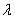on a suitable interval. The associated Green’s function is first given.

2: Paper Source PDF document

Paper's Title:

Boundedness for Vector-Valued Multilinear Singular Integral Operators on Triebel-Lizorkin Spaces

Author(s):

Liu Lanzhe

College of Mathematics
Changsha University of Science and Technology,
Changsha 410077,
P.R. of China.
lanzheliu@263.net

Abstract:

In this paper, the boundedness for some vector-valued multilinear operators associated to certain fractional singular integral operators on Triebel-Lizorkin space are obtained. The operators include Calderón-Zygmund singular integral operator and fractional integral operator.

2: Paper Source PDF document

Paper's Title:

On the Optimal Buckling Loads of Clamped Columns

Author(s):

Samir Karaa

Department of Mathematics and Statistics
Sultan Qaboos University, P.O. Box 36, Alkhod 123
Muscat, Sultanate of Oman
skaraa@squ.edu.om

URL: http://ajmaa.org/EditorsU/SKaraa.php

Abstract:

We consider the problem of determining the optimal shape of a clamped column of given length and volume, without minimum cross section constraints. We prove that the necessary condition of optimality derived by Olhoff and Rasmussen is sufficient when 0<α<1. The number alpha appears in Equation 2.1. For the case α =1 it is shown that the value 48 is optimal. We also determine the exact values of the optimal shape at the extremities, and take advantage of a robust nonlinear ordinary differential equation solver COLSYS to compute the optimal buckling load with a high accuracy.

2: Paper Source PDF document

Paper's Title:

A New Family of Periodic Functions as Explicit Roots of a Class of Polynomial Equations

Author(s):

M. Artzrouni

Department of Mathematics, University of Pau
64013 Pau Cedex
Pau, France
marc.artzrouni@univ-pau.fr
URL: http://www.univ-pau.fr/~artzroun

Abstract:

For any positive integer n a new family of periodic functions in power series form and of period n is used to solve in closed form a class of polynomial equations of order n. The n roots are the values of the appropriate function from that family taken at 0, 1, ... , n-1.

2: Paper Source PDF document

Paper's Title:

Generalizations of Hermite-Hadamard's Inequalities for Log-Convex Functions

Author(s):

Ai-Jun Li

School of Mathematics and Informatics, Henan Polytechnic University,
Jiaozuo City, Henan Province,
454010, China.
liaijun72@163.com

Abstract:

In this article, Hermite-Hadamard's inequalities are extended in terms of the weighted power mean and log-convex function. Several refinements, generalizations and related inequalities are obtained.

2: Paper Source PDF document

Paper's Title:

On the Numerical Solution for Deconvolution Problems with Noise

Author(s):

N. H. Sweilam

Cairo University, Faculty of Science, Mathematics Department,
Giza, Egypt.
n-sweilam@yahoo.com

Abstract:

In this paper three different stable methods for solving numerically deconvolution problems with noise are studied. The methods examined are the variational regularization method, the dynamical systems method, and the iterative regularized processes. Gravity surveying problem with noise is studied as a model problem. The results obtained by these methods are compared to the exact solution for the model problem. It is found that these three methods are highly stable methods and always converge to the solution even for large size models. The relative higher accuracy is obtained by using the iterative regularized processes.

2: Paper Source PDF document

Paper's Title:

On the Asymptotic Behavior of Solutions of Third Order Nonlinear Differential Equations

Author(s):

Ivan Mojsej and Alena Tartaľová

Institute of Mathematics,
Faculty of Science, P. J. Šafárik University,
Jesenná 5, 041 54 Košice,
Slovak Republic
ivan.mojsej@upjs.sk

Department of Applied Mathematics and Business Informatics,
Faculty of Economics, Technical University,
Nemcovej 32, 040 01 Košice,
Slovak Republic
alena.tartalova@tuke.sk

Abstract:

This paper is concerned with the asymptotic behavior of solutions of nonlinear differential equations of the third order with quasiderivatives. Mainly, we present the necessary and sufficient conditions for the existence of nonoscillatory solutions with specified asymptotic behavior as t tends to infinity. These conditions are presented as integral criteria and involve only the coefficients of investigated differential equations. In order to prove some of the results, we use a topological approach based on the Schauder fixed point theorem.

2: Paper Source PDF document

Paper's Title:

Fekete-Szegö Problem for Univalent Functions with Respect to k-Symmetric Points

Author(s):

K. Al-Shaqsi and M. Darus

School of Mathematical Sciences, Faculty of Science and Technology,
University Kebangsaan Malaysia,
Bangi 43600 Selangor D. Ehsan,
Malaysia
ommath@hotmail.com
maslina@ukm.my

Abstract:

In the present investigation, sharp upper bounds of |a3- μa22| for functions f(z) = z + a2z2 + a2z3 + ... belonging to certain subclasses of starlike and convex functions with respect to k-symmetric points are obtained. Also certain applications of the main results for subclasses of functions defined by convolution with a normalized analytic function are given. In particular, Fekete- Szegö inequalities for certain classes of functions defined through fractional derivatives are obtained.

2: Paper Source PDF document

Paper's Title:

Integer Sums of Powers of Trigonometric Functions (MOD p), for prime p

Author(s):

G. J. Tee

Department of Mathematics, University of Auckland, Auckland,
New Zealand
tee@math.auckland.ac.nz

Abstract:

Many multi--parameter families of congruences (mod p) are found for integer sums of  qth powers of the trigonometric functions over various sets of equidistant arguments, where p is any prime factor of q. Those congruences provide sensitive tests for the accuracy of software for evaluating trigonometric functions to high precision.

2: Paper Source PDF document

Paper's Title:

Strongly Nonlinear Variational Parabolic Problems in Weighted Sobolev Spaces

Author(s):

L. Aharouch, E. Azroul and M. Rhoudaf

Dép. Math. Faculté des Sciences Dhar-Mahraz
B.P 1796 Atlas Fés,
Maroc.
rhoudaf_mohamed@yahoo.fr

Abstract:

In this paper , we study the existence of a weak solutions for the initial-boundary value problems of the strongly nonlinear degenerated parabolic equation,

 ∂u +A(u)+g(x,t,u,∇ u)=f ∂t

where A is a Leray-lions operator acted from Lp(0,T,W01,p(Ώ,w)) into its dual. g(x,t,u,∇ u) is a nonlinear term with critical growth condition with respect to ∇ u and no growth with respect to u. The source term f is assumed to belong to Lp'(0,T,W-1,p'(Ώ,w*)).

2: Paper Source PDF document

Paper's Title:

Purely Unrectifiable Sets with Large Projections

Author(s):

Harold R. Parks

Department of Mathematics, Oregon State University,
Corvallis, Oregon 97331--4605,
USA
parks@math.oregonstate.edu
URL
: http://www.math.oregonstate.edu/people/view/parks/

Abstract:

For n2, we give a construction of a compact subset of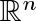that is dispersed enough that it is purely unrectifiable, but that nonetheless has an orthogonal projection that hits every point of an (n-1)-dimensional unit cube. Moreover, this subset has the additional surprising property that the orthogonal projection onto any straight line inis a set of positive 1-dimensional Hausdorff measure.

2: Paper Source PDF document

Paper's Title:

A Study of the Effect of Density Dependence in a Matrix Population Model

Author(s):

N. Carter and M. Predescu

Department of Mathematical Sciences,
Bentley University,
Waltham, MA 02452,
U.S.A.
ncarter@bentley.edu
mpredescu@bentley.edu

Abstract:

We study the behavior of solutions of a three dimensional discrete time nonlinear matrix population model. We prove results concerning the existence of equilibrium points, boundedness, permanence of solutions, and global stability in special cases of interest. Moreover, numerical simulations are used to compare the dynamics of two main forms of the density dependence function (rational and exponential).

2: Paper Source PDF document

Paper's Title:

Improvement of Jensen's Inequality for Superquadratic Functions

Author(s):

S. Abramovich, B. Ivanković, and J. Pečarić

Department of Mathematics,
University of Haifa,
Haifa 31905,
Israel.
abramos@math.haifa.ac.il

Faculty of Transport and Trafic Engineering,
University of Zagreb,
Vukelićeva 4, 10000,
Croatia
bozidar.ivankovic@zg.t-com.hr

Faculty of Textile,
University of Zagreb,
Prilaz Baruna Filipovića 30, 10000 Zagreb,
Croatia
pecaric@element.hr

Abstract:

Since 1907, the famous Jensen's inequality has been refined in different manners. In our paper, we refine it applying superquadratic functions and separations of domains for convex functions. There are convex functions which are not superquadratic and superquadratic functions which are not convex. For superquadratic functions which are not convex we get inequalities analogue to inequalities satisfied by convex functions. For superquadratic functions which are convex (including many useful functions) we get refinements of Jensen's inequality and its extensions.

2: Paper Source PDF document

Paper's Title:

Approximation of Common Fixed Points of a Finite Family of Asymptotically Demicontractive Mappings in Banach Spaces

Author(s):

Yuchao Tang, Yong Cai, Liqun Hu and Liwei Liu

Department of Mathematics, NanChang University,
Nanchang 330031, P.R. China
Department of Mathematics, Xi'an Jiaotong University,
Xi'an 710049, P.R. China

hhaaoo1331@yahoo.com.cn

Abstract:

By virtue of new analytic techniques, we analyze and study
several strong convergence theorems for the approximation of
common fixed points of asymptotically demicontractive mappings
via the multistep iterative sequence with errors in Banach
spaces. Our results improve and extend the corresponding ones
announced by Osilike , Osilike and Aniagbosor, Igbokwe, Cho et
al., Moore and Nnoli, Hu and all the others.

2: Paper Source PDF document

Paper's Title:

Sharp Lp Improving Results for Singular Measures on Cn+1

Author(s):

E. Ferreyra, M. Urciuolo

FaMAF-CIEM,
Argentina

Abstract:

For j=1,...,n, let Ωj be open sets of the complex plane and let φj be holomorphic functions on Ωj such that φj'' does not vanish identically on Ωj. We consider φ(z1,...,zn) =φ1(z1) +...+φn(zn). We characterize the pairs (p,q) such that the convolution operator with the surface measure supported on a compact subset of the graph of φ is p-q bounded.

2: Paper Source PDF document

Paper's Title:

Ulam Stability of Reciprocal Difference and Adjoint Functional Equations

Author(s):

K. Ravi, J. M. Rassias and B. V. Senthil Kumar

Department of Mathematics,
Sacred Heart College, Tirupattur - 635601,
India

Pedagogical Department E. E.,
Section of Mathematics and Informatics,
National and Capodistrian University of Athens,
Athens, Attikis 15342,
GREECE

Department of Mathematics,
C.Abdul Hakeem College of Engineering and
Technology, Melvisharam - 632 509, India

shckavi@yahoo.co.in
jrassias@primedu.uoa.gr
bvssree@yahoo.co.in

Abstract:

In this paper, the reciprocal difference functional equation (or RDF equation) and the reciprocal adjoint functional equation (or RAF equation) are introduced. Then the pertinent Ulam stability problem for these functional equations is solved, together with the extended Ulam (or Rassias) stability problem and the generalized Ulam (or Ulam-Gavruta-Rassias) stability problem for the same equations.

2: Paper Source PDF document

Paper's Title:

On Generalization of Hardy-type Inequalities

Author(s):

K. Rauf, S. Ponnusamy and J. O. Omolehin

Department of Mathematics,
University of Ilorin, Ilorin,
Nigeria
krauf@unilorin.edu.ng

Department of Mathematics,
Chennai- 600 036,
India
samy@iitm.ac.in

Department of Mathematics,
University of Ilorin, Ilorin,
Nigeria
omolehin_joseph@yahoo.com

Abstract:

This paper is devoted to some new generalization of Hardy-type integral inequalities and the reversed forms. The study is to determine conditions on which the generalized inequalities hold using some known hypothesis. Improvement of some inequalities are also presented.

2: Paper Source PDF document

Paper's Title:

On Convergence Theorems of an Implicit Iterative Process with Errors for a Finite Family of Asymptotically quasi I-nonexpansive Mappings

Author(s):

Farrukh Mukhamedov and Mansoor Saburov

Department of Computational & Theoretical Sciences,
Faculty of Sciences, International Islamic University Malaysia,
P.O. Box, 141, 25710, Kuantan,
Malaysia

farrukh_m@iiu.edu.my

msaburov@gmail.com

http://www.iium.edu.my

Abstract:

In this paper we prove the weak and strong convergence of the implicit iterative process with errors to a common fixed point of a finite family {Tj}Ni=1 of asymptotically quasi Ij-nonexpansive mappings as well as a family of {Ij}Nj=1 of asymptotically quasi nonexpansive mappings in the framework of Banach spaces. The obtained results improve and generalize the corresponding results in the existing literature.

2: Paper Source PDF document

Paper's Title:

New Inequalities of Mill's Ratio and Application to The Inverse Q-function Approximation

Author(s):

Pingyi Fan

Department of Electronic Engineering,
Tsinghua University, Beijing,
China

fpy@tsinghua.edu.cn

Abstract:

In this paper, we investigate the Mill's ratio estimation problem and get two new inequalities. Compared to the well known results obtained by Gordon, they becomes tighter. Furthermore, we also discuss the inverse Q-function approximation problem and present some useful results on the inverse solution. Numerical results confirm the validness of our theoretical analysis. In addition, we also present a conjecture on the bounds of inverse solution on Q-function.

2: Paper Source PDF document

Paper's Title:

Numerical Solution of A System of Singularly Perturbed Convection-Diffusion Boundary-Value Problems Using Mesh Equidistribution Technique

Author(s):

Pratibhamoy Das and Srinivasan Natesan

Department of Mathematics,
Indian Institute of Technology Guwahati,
Guwahati - 781 039,
India.

pratibhamoy@gmail.com
natesan@iitg.ernet.in
URL: http://www.iitg.ernet.in/natesan/

Abstract:

In this article, we consider a system of singularly perturbed weakly coupled convection-diffusion equations having diffusion parameters of different magnitudes. These small parameters give rise to boundary layers. An upwind finite difference scheme on adaptively generated mesh is used to obtain a suitable monitor function that gives first-order convergence which is robust with respect to the diffusion parameters. We present the results of numerical experiments for linear and semilinear system of differential equations to support the effectiveness of our preferred monitor function obtained from theoretical analysis.

2: Paper Source PDF document

Paper's Title:

Application of Equivalence Method to Classify Monge-Ampčre Equations of Elliptic Type

Author(s):

Moheddine Imsatfia

E-mail: imsatfia@math.jussieu.fr

Abstract:

In this paper, we apply Cartan's equivalence method to give a local classification of Monge-Ampčre equations of elliptic type. Then we find a necessary and sufficient conditions such that a Monge-Ampčre equation is either contactomorphic to the Laplace equation or to an Euler-Lagrange equation.

2: Paper Source PDF document

Paper's Title:

Partial Semigroup Algebras Associated to Partial Action

Author(s):

Bahman Tabatabaie Shourijeh and Sahar Moayeri Rahni

Department of Mathematics,
College of Sciences, Shiraz University,
Shiraz, 71454,
Iran.
E-mail: tabataba@math.susc.ac.ir

Department of Mathematics,
College of Sciences, Shiraz University,
Shiraz, 71454,
Iran.
E-mail: smoayeri@shirazu.ac.ir

Abstract:

For a given inverse semigroup S, we introduce the notion of algebraic crossed product by using a given partial action of S, and we will prove that under some condition it is associative. Also we will introduce the concept of partial semigroup algebra KPar(S), and we show that the suitable quotient of KPar(S) is a kind of crossed product.

2: Paper Source PDF document

Paper's Title:

Stability of the D-Bar Reconstruction Method for Complex Conductivities

Author(s):

1S. El Kontar, 1T. El Arwadi, 1H. Chrayteh, 2J.-M. Sac-Épée

1Department of Mathematics and Computer Science,
Faculty of Science, Beirut Arab University,
P.O. Box: 11-5020, Beirut,
Lebanon.

E-mail: srs915@student.bau.edu.lb

2Institut Élie Cartan de Lorraine,
Université de Lorraine - Metz,
France.

Abstract:

In 2000, Francini solved the inverse conductivity problem for twice-differentiable conductivities and permittivities. This solution was considered to be the first approach using D-bar methods with complex conductivities. In 2012, based on Francini's work, Hamilton introduced a reconstruction method of the conductivity distribution with complex values. The method consists of six steps. A voltage potential is applied on the boundary. Solving a D-Bar equation gives the complex conductivity. In this paper, the stability of the D-Bar equation is studied via two approximations, texp and tB, for the scattering transform. The study is based on rewriting the reconstruction method in terms of continuous operators. The conductivity is considered to be non smooth.

2: Paper Source PDF document

Paper's Title:

Some New Inequalities of Hermite-Hadamard and Fejér Type for Certain Functions with Higher Convexity

Author(s):

Steven G. From

Department of Mathematics,
U.S.A.
E-mail: sfrom@unomaha.edu

Abstract:

In this paper, we present some new inequalities of Hermite-Hadamard or Fejér type for certain functions satisfying some higher convexity conditions on one or more derivatives.
An open problem is given also.
Some applications to the logarithmic mean are given.

2: Paper Source PDF document

Paper's Title:

Convergence Speed of Some Random Implicit-Kirk-type Iterations for Contractive-type Random Operators

Author(s):

H. Akewe, K.S. Eke

Department of Mathematics,
Covenant University,
Canaanland, KM 10, Idiroko Road, P. M. B. 1023, Ota, Ogun State,
Nigeria.
E-mail: hudson.akewe@covenantuniversity.edu.ng, kanayo.eke@covenantuniversity.edu.ng

Abstract:

The main aim of this paper is to introduce a stochastic version of multistep type iterative scheme called a modified random implicit-Kirk multistep iterative scheme and prove strong convergence and stability results for a class of generalized contractive-type random operators. The rate of convergence of the random iterative schemes are also examined through an example. The results show that our new random implicit kirk multistep scheme perform better than other implicit iterative schemes in terms of convergence and thus have good potentials for further applications in equilibrium problems in computer science, physics and economics.

2: Paper Source PDF document

Paper's Title:

The Influence of Fluid Pressure in Macromechanical Cochlear Model

Author(s):

F. E. Aboulkhouatem1, F. Kouilily1, N. Achtaich1, N. Yousfi1 and M. El Khasmi2

1Department of Mathematics and Computer Science, Faculty of Sciences
Ben M'sik, Hassan II University, Casablanca,
Morocco.

2Department of Biology, Faculty of Sciences
Ben M'sik, Hassan II University, Casablanca,
Morocco.

E-mail: fatiaboulkhouatem@gemail.com
URL: http://www.fsb.univh2c.ma/

Abstract:

An increase of pressure in the structure of cochlea may cause a hearing loss. In this paper, we established the relationship between the fluid pressure and the amplitude of displacement of Basilar Membrane to clarify the mechanisms of hearing loss caused by increasing of this pressure. So, a mathematical cochlear model was formulated using finite difference method in order to explain and demonstrate this malfunction in passive model. Numerical simulations may be considered as helpful tools which may extend and complete the understanding of a cochlea dysfunction.

2: Paper Source PDF document

Paper's Title:

Cubic Alternating Harmonic Number Sums

Author(s):

Anthony Sofo

Victoria University,
College of Engineering and Science,
Melbourne City,
Australia.
E-mail: Anthony.Sofo@vu.edu.au

Abstract:

We develop new closed form representations of sums of cubic alternating harmonic numbers and reciprocal binomial coefficients. We also identify a new integral representation for the ζ (4)  constant.

2: Paper Source PDF document

Paper's Title:

On an extension of Edwards's double integral with applications

Author(s):

I. Kim, S. Jun, Y. Vyas and A. K. Rathie

Department of Mathematics Education,
Wonkwang University,
Iksan, 570-749,
Republic of Korea.

General Education Institute,
Konkuk University,
Chungju 380-701,
Republic of Korea.

Department of Mathematics, School of Engineering,
Bhatewar, Udaipur, 313601, Rajasthan State,
India.

Department of Mathematics,
Vedant College of Engineering and Technology,
(Rajasthan Technical University),
Bundi-323021, Rajasthan,
India.

E-mail: iki@wku.ac.kr sjun@kku.ac.kr
yashoverdhan.vyas@spsu.ac.in arjunkumarrathie@gmail.com

Abstract:

The aim of this note is to provide an extension of the well known and useful Edwards's double integral. As an application, new class of twelve double integrals involving hypergeometric function have been evaluated in terms of gamma function. The results are established with the help of classical summation theorems for the series 3F2 due to Watson, Dixon and Whipple. Several new and interesting integrals have also been obtained from our main findings.

2: Paper Source PDF document

Paper's Title:

The Concept of Convergence for 2-Dimensional Subspaces Sequence in Normed Spaces

Author(s):

M. Manuharawati, D. N. Yunianti, M. Jakfar

Mathematics Department, Universitas Negeri Surabaya,
Jalan Ketintang Gedung C8,
Surabaya 60321,
Indonesia.
E-mail: manuharawati@unesa.ac.id, dwiyunianti@unesa.ac.id,

Abstract:

In this paper, we present a concept of convergence of sequence, especially, of 2-dimensional subspaces of normed spaces. The properties of the concept are established. As consequences of our definition in an inner product space, we also obtain the continuity property of the angle between two 2-dimensional subspaces of inner product spaces.

2: Paper Source PDF document

Paper's Title:

Bounds on the Jensen Gap, and Implications for Mean-Concentrated Distributions

Author(s):

Xiang Gao, Meera Sitharam, Adrian E. Roitberg

Department of Chemistry, and Department of Computer & Information Science & Engineering,
University of Florida,
Gainesville, FL 32611,
USA.
E-mail: qasdfgtyuiop@gmail.com

Abstract:

This paper gives upper and lower bounds on the gap in Jensen's inequality, i.e., the difference between the expected value of a function of a random variable and the value of the function at the expected value of the random variable. The bounds depend only on growth properties of the function and specific moments of the random variable. The bounds are particularly useful for distributions that are concentrated around the mean, a commonly occurring scenario such as the average of i.i.d. samples and in statistical mechanics.

2: Paper Source PDF document

Paper's Title:

Composite Variational-Like Inequalities Given By Weakly Relaxed ζ-Semi-Pseudomonotone Multi-Valued Mapping

Author(s):

Syed Shakaib Irfan, Iqbal Ahmad, Zubair Khan and Preeti Shukla

College of Engineering, Qassim University
Buraidah, Al-Qassim,
Saudi Arabia.
E-mail: shakaib@qec.edu.sa

College of Engineering, Qassim University
Buraidah, Al-Qassim,
Saudi Arabia.
E-mail: iqbal@qec.edu.sa

Department of Mathematics,
Integral University Lucknow,
India.
E-mail: zkhan@iul.ac.in

Department of Mathematics,
Integral University Lucknow,
India.
E-mail: shuklapreeti1991@gmail.com

Abstract:

In this article, we introduce a composite variational-like inequalities with weakly relaxed ζ-pseudomonotone multi-valued maping in reflexive Banach spaces. We obtain existence of solutions to the composite variational-like inequalities with weakly relaxed ζ-pseudomon -otone multi-valued maps in reflexive Banach spaces by using KKM theorem. We have also checked the solvability of the composite variational-like inequalities with weakly relaxed ζ-semi-pseudomonotone multi-valued maps in arbitrary Banach spaces using Kakutani-Fan-Glicksberg fixed point theorem.

1: Paper Source PDF document

Paper's Title:

Asymptotic Behavior of Mixed Type Functional Equations

Author(s):

J. M. Rassias

Pedagogical Department, E.E., National and Capodistrian University of Athens, Section of Mathematics And Informatics, 4, Agamemnonos Str., Aghia Paraskevi, Athens 15342,Greece
jrassias@primedu.uoa.gr
URL: http://www.primedu.uoa.gr/~jrassias/

Abstract:

In 1983 Skof  was the first author to solve the Ulam problem for additive mappings on a restricted domain. In 1998 Jung  investigated the Hyers-Ulam stability of additive and quadratic mappings on restricted domains. In this paper we improve the bounds and thus the results obtained by Jung , in 1998 and by the author , in 2002. Besides we establish new theorems about the Ulam stability of mixed type functional equations on restricted domains. Finally, we apply our recent results to the asymptotic behavior of functional equations of different types.

1: Paper Source PDF document

Paper's Title:

An Algorithm to Compute Gaussian-Type Quadrature Formulae
that Integrate Polynomials and Some Spline Functions Exactly

Author(s):

Allal Guessab

Laboratoire de Mathématiques Appliquées,
Université de Pau, 64000, Pau,
France.
allal.guessab@univ-pau.fr
URL: http://www.univ-pau.fr/~aguessab/

Abstract:

It is well-known that for sufficiently smooth integrands on an interval, numerical integration can be performed stably and efficiently via the classical (polynomial) Gauss quadrature formulae. However, for many other sets of integrands these quadrature formulae do not perform well. A very natural way of avoiding this problem is to include a wide class among arbitrary functions (not necessary polynomials) to be integrated exactly. The spline functions are natural candidates for such problems. In this paper, after studying Gaussian type quadrature formulae which are exact for spline functions and which contain boundary terms involving derivatives at both end points, we present a fast algorithm for computing their nodes and weights. It is also shown, taking advantage of the close connection with ordinary Gauss quadrature formula, that the latter are computed, via eigenvalues and eigenvectors of real symmetric tridiagonal matrices. Hence a new class of quadrature formulae can then be computed directly by standard software for ordinary Gauss quadrature formula. Comparative results with classical Gauss quadrature formulae are given to illustrate the numerical performance of the approach.

1: Paper Source PDF document

Paper's Title:

Error estimates for aproximations of the Fourier transform of functions in Lp spaces

Author(s):

A. Aglic Aljinovic and J. Pecaric

Department of Applied Mathematics,
Faculty of Electrical Engineering and Computing,
University Of Zagreb, Unska 3, 10 000 Zagreb,
Croatia
andrea@zpm.fer.hr

Faculty of Textile Technology,
University of Zagreb, Pierottijeva 6, 10000 Zagreb,
Croatia
pecaric@hazu.hr

Abstract:

New inequalities concerning the Fourier transform are given. Estimates of the difference between two Fourier transforms and even bounds to the associated numerical quadrature formulae are provided as well.

1: Paper Source PDF document

Paper's Title:

On Certain Classes of Harmonic Univalent Functions Based on Salagean Operator

Author(s):

G. Murugusundaramoorthy, Thomas Rosy, and B. A. Stephen

Department of Applied Mathematics and Informatics,
Department of Mathematics, Vellore Institute of Technology,
Deemed University, Vellore - 632014, India.
gmsmoorthy@yahoo.com

Department of Applied Mathematics and Informatics,
Department of Mathematics, Madras Christian College,
Chennai - 600059, India.
drthomasrosy@rediffmail.com

Abstract:

We define and investigate a class of complex-valued harmonic univalent functions of the form f = h + g using Salagean operator where h and g are analytic in the unit disc U = { z : |z| < 1 }. A necessary and sufficient coefficient conditions are given for functions in these classes. Furthermore, distortion theorems, inclusion relations, extreme points, convolution conditions and convex combinations for this family of harmonic functions are obtained.

1: Paper Source PDF document

Paper's Title:

On the Ulam Stability for Euler-Lagrange Type Quadratic Functional Equations

Author(s):

Matina John Rassias and John Michael Rassias

Statistics and Modelling Science,
University of Strathclyde,
Livingstone Tower,
26 Richmond Str,
Glasgow, Uk, G1 1xh

Pedagogical Department, E. E., National and Capodistrian University of Athens,
Section of Mathematics and Informatics,
Athens 15342, Greece

Abstract:

In 1940 (and 1968) S. M. Ulam proposed the well-known Ulam stability problem. In 1941 D.H. Hyers solved the Hyers-Ulam problem for linear mappings. In 1951 D. G. Bourgin has been the second author treating the Ulam problem for additive mappings. In 1978 according to P.M. Gruber this kind of stability problems is of particular interest in probability theory and in the case of functional equations of different types. In 1982-2004 we established the Hyers-Ulam stability for the Ulam problem for different mappings. In 1992-2000 J.M. Rassias investigated the Ulam stability for Euler-Lagrange mappings. In this article we solve the Ulam problem for Euler-Lagrange type quadratic functional equations. These stability results can be applied in mathematical statistics, stochastic analysis, algebra, geometry, as well as in psychology and sociology.

1: Paper Source PDF document

Paper's Title:

New Coincidence and Fixed Point Theorems for Strictly Contractive Hybrid Maps

Author(s):

S. L. Singh and Amal M. Hashim

21, Govind Nagar, Rishikesh 249201,
Ua, India
vedicmri@sancharnet.in

Dept. of Math., College of Science,
Univ. of Basarah,
Iraq.

Abstract:

The purpose of this paper is to study the (EA)-property and noncompatible maps of a hybrid pair of single-valued and multivalued maps in fixed point considerations. Such maps have the remarkable property that they need not be continuous at their common fixed points. We use this property to obtain some coincidence and fixed point theorems for strictly contractive hybrid maps without using their continuity and completeness or compactness of the space.

1: Paper Source PDF document

Paper's Title:

Essential Random Fixed Point Set of Random Operators

Author(s):

Ismat Beg

Centre for Advanced Studies in Mathematics,
Lahore University of Management Sciences (LUMS),
54792-Lahore, PAKISTAN. ibeg@lums.edu.pk
URL: http://web.lums.edu.pk/~ibeg

Abstract:

We obtain necessary and sufficient conditions for the existence of essential random fixed point of a random operator defined on a compact metric space. The structure of the set of essential random fixed points is also studied.

1: Paper Source PDF document

Paper's Title:

Solution of the Hyers-Ulam Stability Problem for Quadratic Type Functional Equations in Several Variables

Author(s):

John Michael Rassias

Pedagogical Department, E.E., National and Capodistrian University of Athens,
Section of Mathematics and Informatics,
Athens 15342,
Greece jrassias@primedu.uoa.gr
URL: http://www.primedu.uoa.gr/~jrassias/

Abstract:

In 1940 (and 1968) S. M. Ulam proposed the well-known Ulam stability problem. In 1941 D. H. Hyers solved the Hyers-Ulam problem for linear mappings. In 1951 D. G. Bourgin has been the second author treating the Ulam problem for additive mappings. In 1978 according to P. M. Gruber this kind of stability problems is of particular interest in probability theory and in the case of functional equations of different types. In 1982-2004 we established the Hyers-Ulam stability for the Ulam problem for different mappings. In this article we solve the Hyers-Ulam problem for quadratic type functional equations in several variables. These stability results can be applied in stochastic analysis, financial and actuarial mathematics, as well as in psychology and sociology.

1: Paper Source PDF document

Paper's Title:

On Perturbed Reflection Coefficients

Author(s):

J. L. Díaz-Barrero and J. J. Egozcue

Applied Mathematics III,
Barcelona, Spain
jose.luis.diaz@upc.edu
juan.jose.egozcue@upc.edu

Abstract:

Many control and signal processing applications require testing stability of polynomials. Classical tests for locating zeros of polynomials are recursive, but they must be stopped whenever the so called "singular polynomials" appear. These ``singular cases'' are often avoided by perturbing the "singular polynomial". Perturbation techniques although always successful are not proven to be well-founded. Our aim is to give a mathematical foundation to a perturbation method in order to overcome "singular cases" when using Levinson recursion as a testing method. The non-singular polynomials are proven to be dense in the set of all polynomials respect the L˛-norm on the unit circle . The proof is constructive and can be used algorithmically.

1: Paper Source PDF document

Paper's Title:

A New Hardy-Hilbert's Type Inequality for Double Series and its Applications

Author(s):

Mingzhe Gao

Department of Mathematics and Computer Science, Normal College Jishou University,
Jishou Hunan, 416000,
People's Republic of China
mingzhegao1940@yahoo.com.cn

Abstract:

In this paper, it is shown that a new Hardy-Hilbert’s type inequality for double series can be established by introducing a parameter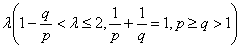and the weight function of the form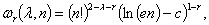where c is Euler constant and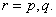And the coefficient is proved to be the best possible. And as the mathematics aesthetics, several important constants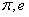and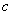appear simultaneously in the coefficient and the weight function when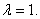In particular, for case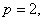some new Hilbert’s type inequalities are obtained. As applications, some extensions of Hardy-Littlewood’s inequality are given.

1: Paper Source PDF document

Paper's Title:

Existence of Non-spurious Solutions to Discrete Boundary Value Problems

Author(s):

Irena Rachunkova and Christopher C. Tisdell

Department of Mathematics
Palacky University
771 46 Olomouc, Czech Republic.
rachunko@risc.upol.cz
URL: http://phoenix.inf.upol.cz/~rachunekl/mathair/matha-en.htm

School of Mathematics
The University of New South Wales
Sydney 2052, Australia.
cct@unsw.edu.au
URL: http://www.maths.unsw.edu.au/~cct

Abstract:

This paper investigates discrete boundary value problems (BVPs) involving second-order difference equations and two-point boundary conditions. General theorems guaranteeing the existence and uniqueness of solutions to the discrete BVP are established. The methods involve a sufficient growth condition to yield an a priori bound on solutions to a certain family of discrete BVPs. The em a priori bounds on solutions to the discrete BVP do not depend on the step-size and thus there are no ``spurious'' solutions. It is shown that solutions of the discrete BVP will converge to solutions of ordinary differential equations.

1: Paper Source PDF document

Paper's Title:

On the Fekete-Szegő Inequality for Some Subclasses of Analytic Functions

Author(s):

T.N. Shanmugam and A. Singaravelu

Department of Mathematics,
College of Engineering,
Anna University, Chennai-600 025,
shan@annauniv.edu

Department of Mathematics,
Valliammai Engineering College,
Chennai-603 203,
sivasaisastha@rediffmail.com

Abstract:

In this present investigation, the authors obtainFekete-Szegő's inequality for certain normalized analytic functions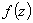defined on the open unit disk for which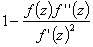lie in a region starlike with respect to 1 and symmetric with respect to the real axis. Also certain applications of the main result for a class of functions defined by convolution are given. As a special case of this result, Fekete-Szegő's inequality for a class of functions defined through fractional derivatives is also obtained.

1: Paper Source PDF document

Paper's Title:

A Coefficient Inequality For Certain Subclasses of Analytic Functions Related to Complex Order

Author(s):

B. Srutha Keerthi, B. Adolf Stephen and S. Sivasubramanian

Department Of Applied Mathematics, Sri Venkateswara College Of Engineering, Anna University,
Sriperumbudur, Chennai - 602 105,
India.
laya@svce.ac.in

Department of Mathematics, Madras Christian College, Chennai - 600059,
India

Department of Mathematics, College of Engineering, Anna University,
India.
sivasaisastha@rediffmail.com

Abstract:

In this present investigation, the authors obtain coefficient inequality for certain normalized analytic functions of complex order f(z) defined on the open unit disk for which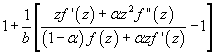(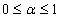and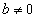be a complex number) lies in a region starlike with respect to 1 and is symmetric with respect to the real axis. Also certain applications of the main result for a class of functions of complex order defined by convolution are given. As a special case of this result, coefficient inequality for a class of functions defined through fractional derivatives is obtained. The motivation of this paper is to give a generalization of the coefficient inequalities of the subclasses of starlike and convex functions of complex order.

1: Paper Source PDF document

Paper's Title:

Product Formulas Involving Gauss Hypergeometric Functions

Author(s):

Edward Neuman

Department of Mathematics, Mailcode 4408,
Southern Illinois University,
1245 Lincoln Drive,
Carbondale, IL 62901,
USA.
edneuman@math.siu.edu
URL: http://www.math.siu.edu/neuman/personal.html

Abstract:

New formulas for a product of two Gauss hypergeometric functions are derived. Applications to special functions, with emphasis on Jacobi polynomials, Jacobi functions, and Bessel functions of the first kind, are included. Most of the results are obtained with the aid of the double Dirichlet average of a univariate function.

1: Paper Source PDF document

Paper's Title:

Construction of Lyapunov Functionals In Functional Differential Equations With Applications To Exponential Stability In Volterra Integro-differential Equations

Author(s):

Youssef N. Raffoul

Department of Mathematics, University of Dayton,
Dayton OH 45469-2316,
USA
youssef.raffoul@notes.udayton.edu

Abstract:

Non-negative definite Lyapunov functionals are employed to obtain sufficient conditions that guarantee the exponential asymptotic stability and uniform exponential asymptotic stability of the zero solution of nonlinear functional differential systems. The theory is applied to Volterra integro-differential equations in the form of proposition examples.

1: Paper Source PDF document

Paper's Title:

On an Integral Inequality of the Hardy-Type

Author(s):

C. O. Imoru and A. G. Adeagbo-Sheikh

Department of Mathematics
Obafemi Awolowo University, Ile-Ife, Nigeria.
cimoru@oauife.edu.ng
asheikh@oauife.edu.ng

Abstract:

In this paper, we obtain an integral inequality which extends Shum's and Imoru's generalization of Hardy's Inequality. Our main tool is Imoru's adaptation of Jensen's Inequality for convex functions.

1: Paper Source PDF document

Paper's Title:

Normalized Truncated Levy models applied to the study of Financial Markets

Author(s):

M. C. Mariani, K. Martin, D. W. Dombrowski and D. Martinez

Department of Mathematical Sciences and Department of Finance,
New Mexico State University, P.O. Box 30001
Department 3MB Las Cruces, New Mexico 88003-8001
USA.
mmariani@nmsu.edu
kjmartin@nmsu.edu

Abstract:

This work is devoted to the study of the statistical properties of financial instruments from developed markets. We performed a new analysis of the behavior of companies corresponding to the DJIA index, and of the index itself, by using a normalized Truncated Levy walk model. We conclude that the Truncated Levy distribution describes perfectly the evolution of the companies and of the index near a crash.

1: Paper Source PDF document

Paper's Title:

On Some Remarkable Product of Theta-function

Author(s):

M. S. Mahadeva Naika, M. C. Maheshkumar and K. Sushan Bairy

Department of Mathematics,
Bangalore University, Central College Campus,
Bangalore-560 001,
INDIA
msmnaika@rediffmail.com
softmahe@rediffmail.com
ksbairy@gmail.com

Abstract:

On pages 338 and 339 in his first notebook, Ramanujan records eighteen values for a certain product of theta-function. All these have been proved by B. C. Berndt, H. H. Chan and L-C. Zhang . Recently M. S. Mahadeva Naika and B. N. Dharmendra [7, 8] and Mahadeva Naika and M. C. Maheshkumar  have obtained general theorems to establish explicit evaluations of Ramanujan's remarkable product of theta-function. Following Ramanujan we define a new function bM,N as defined in (1.5). The main purpose of this paper is to establish some new general theorems for explicit evaluations of product of theta-function.

1: Paper Source PDF document

Paper's Title:

Equilibria and Periodic Solutions of Projected Dynamical Systems on Sets with Corners

Author(s):

Matthew D. Johnston and Monica-Gabriela Cojocaru

Department of Applied Mathematics, University of Waterloo,
mdjohnst@math.uwaterloo.ca

Department of Mathematics & Statistics, University of Guelph,
mcojocar@uoguelph.ca

Abstract:

Projected dynamical systems theory represents a bridge between the static worlds of variational inequalities and equilibrium problems, and the dynamic world of ordinary differential equations. A projected dynamical system (PDS) is given by the flow of a projected differential equation, an ordinary differential equation whose trajectories are restricted to a constraint set K. Projected differential equations are defined by discontinuous vector fields and so standard differential equations theory cannot apply. The formal study of PDS began in the 90's, although some results existed in the literature since the 70's. In this paper we present a novel result regarding existence of equilibria and periodic cycles of a finite dimensional PDS on constraint sets K, whose points satisfy a corner condition. The novelty is due to proving existence of boundary equilibria without using a variational inequality approach or monotonicity type conditions.

1: Paper Source PDF document

Paper's Title:

On the Generalized Stability and Asymptotic Behavior of Quadratic Mappings

Author(s):

Hark-Mahn Kim, Sang-Baek Lee and Eunyoung Son

Department of Mathematics
Chungnam National University
Daejeon, 305-764,
Republic of Korea

hmkim@cnu.ac.kr

Abstract:

We extend the stability of quadratic mappings to the stability of general quadratic mappings with several variables, and then obtain an improved asymptotic property of quadratic mappings on restricted domains.

1: Paper Source PDF document

Paper's Title:

Differentiability of Distance Functions in p-Normed Spaces

Author(s):

M. S. Moslehian, A. Niknam, S. Shadkam Torbati

Department of Pure Mathematics, Centre of Excellence in Analysis on Algebraic Structures (CEAAS),,
Iran

moslehian@ferdowsi.um.ac.ir
niknam@math.um.ac.ir

Abstract:

The farthest point mapping in a p-normed space X is studied in virtue of the Gateaux derivative and the Frechet derivative. Let M be a closed bounded subset of X having the uniformly p-Gateaux differentiable norm. Under certain conditions, it is shown that every maximizing sequence is convergent, moreover, if M is a uniquely remotal set then the farthest point mapping is continuous and so M is singleton. In addition, a Hahn--Banach type theorem in \$p\$-normed spaces is proved.

1: Paper Source PDF document

Paper's Title:

Stability of Almost Multiplicative Functionals

Author(s):

Norio Niwa, Hirokazu Oka, Takeshi Miura and Sin-Ei Takahasi

Faculty of Engineering, Osaka Electro-Communication University,
Neyagawa 572-8530,
Japan

Faculty of Engineering, Ibaraki University,
Hitachi 316-8511,
Japan

Department of Applied Mathematics and Physics, Graduate School of Science and Engineering,
Yamagata University,
Yonezawa 992-8510
Japan

oka@mx.ibaraki.ac.jp
miura@yz.yamagata-u.ac.jp
sin-ei@emperor.yz.yamagata-u.ac.jp

Abstract:

Let δ and p be non-negative real numbers. Let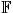be the real or complex number field and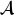a normed algebra over. If a mapping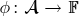satisfies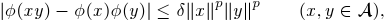then we show that φ is multiplicative or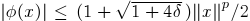for all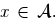If, in addition, φ satisfies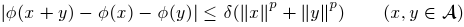for some p1, then by using Hyers-Ulam-Rassias stability of additive Cauchy equation, we show that φ is a ring homomorphism or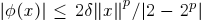for all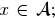In other words, φ is a ring homomorphism, or an approximately zero mapping. The results of this paper are inspired by Th.M. Rassias' stability theorem.

1: Paper Source PDF document

Paper's Title:

Hyperbolic Barycentric Coordinates

Author(s):

Abraham A. Ungar

Department of Mathematics, North Dakota State University,
Fargo, ND 58105,
USA
Abraham.Ungar@ndsu.edu
URL
: http://math.ndsu.nodak.edu/faculty/ungar/

Abstract:

A powerful and novel way to study Einstein's special theory of relativity and its underlying geometry, the hyperbolic geometry of Bolyai and Lobachevsky, by analogies with classical mechanics and its underlying Euclidean geometry is demonstrated. The demonstration sets the stage for the extension of the notion of barycentric coordinates in Euclidean geometry, first conceived by Möbius in 1827, into hyperbolic geometry. As an example for the application of hyperbolic barycentric coordinates, the hyperbolic midpoint of any hyperbolic segment, and the centroid and orthocenter of any hyperbolic triangle are determined.

1: Paper Source PDF document

Paper's Title:

Superquadracity, Bohr's Inequality and Deviation from a Mean Value

Author(s):

S. Abramovich, J. Barić, and J. Pečarić

Department of Mathematics, University of Haifa,
Haifa, 31905,
Israel

abramos@math.haifa.ac.il

FESB, University of Split,
Rudera Bošcovića,
B.B., 21000, Split,
Croatia
jbaric@fesb.hr

Faculty of Textile Technology, University of Zagreb,
Prilaz Baruna Filipovića,
30, 10000 Zagreb,
Croatia.
pecaric@hazu.hr

Abstract:

Extensions of Bohr's inequality via superquadracity are obtained, where instead of the power p=2 which appears in Bohr's inequality we get similar results when we deal with p≥ 2 and with p≤ 2. Also, via superquadracity we extend a bound for deviation from a Mean Value.

1: Paper Source PDF document

Paper's Title:

On some Strongly Nonlinear Elliptic Problems in -data with a Nonlinearity Having a Constant Sign in Orlicz Spaces via Penalization Methods

Author(s):

E. Azroul, A. Benkirane and M. Rhoudaf

Dep. Math., Faculté des Sciences Dhar-Mahraz,
B.P 1796 Atlas Fčs,
Maroc

Departement of Mathematics,
Faculty of Sciences and Techniques of Tangier,
B.P. 416, Tangier,
Morocco.
rhoudaf_mohamed@yahoo.fr

Abstract:

This paper is concerned with the existence result of the unilateral problem associated to the equations of the type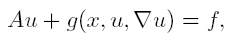in Orlicz spaces, without assuming the sign condition in the nonlinearity g. The source term f belongs to Lą(Ώ).

1: Paper Source PDF document

Paper's Title:

Ellipses of Minimal Area and of Minimal Eccentricity Circumscribed About a Convex Quadrilateral

Author(s):

Alan Horwitz

Penn State University,
25 Yearsley Mill Rd.,
Media, PA 19063,
U.S.A
alh4@psu.edu

Abstract:

First, we fill in key gaps in Steiner's nice characterization of the most nearly circular ellipse which passes through the vertices of a convex quadrilateral,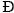. Steiner proved that there is only one pair of conjugate directions, M1 and M2, that belong to all ellipses of circumscription. Then he proves that if there is an ellipse, E, whose equal conjugate diameters possess the directional constants M1 and M2, then E must be an ellipse of circumscription which has minimal eccentricity. However, Steiner does not show the existence or uniqueness of such an ellipse. We prove that there is a unique ellipse of minimal eccentricity which passes through the vertices of. We also show that there exists an ellipse which passes through the vertices ofand whose equal conjugate diameters possess the directional constants M1 and M2. We also show that there exists a unique ellipse of minimal area which passes through the vertices of. Finally, we call a convex quadrilateral,, bielliptic if the unique inscribed and circumscribed ellipses of minimal eccentricity have the same eccentricity. This generalizes the notion of bicentric quadrilaterals. In particular, we show the existence of a bielliptic convex quadrilateral which is not bicentric.

1: Paper Source PDF document

Paper's Title:

Approximation of Derivatives in a Singularly Perturbed Second Order Ordinary Differential Equation with Discontinuous Terms Arising in Chemical Reactor Theory

Author(s):

R. Mythili Priyadharshini and N. Ramanujam

Department of Mathematics, Bharathidasan University,
Tiruchirappalli - 620 024, Tamilnadu, India.
matram2k3@yahoo.com
URL: http://www.bdu.ac.in/depa/science/ramanujam.htm

Abstract:

In this paper, a singularly perturbed second order ordinary differential equation with a discontinuous convection coefficient arising in chemical reactor theory is considered. A robust-layer-resolving numerical method is suggested. An ε-uniform global error estimate for the numerical solution and also to the numerical derivative are established. Numerical results are provided to illustrate the theoretical results.

1: Paper Source PDF document

Paper's Title:

Real Interpolation Methods and Quasilogarithmic Operators

Author(s):

Ming Fan

School of Industrial Technology and Management,
Dalarna University, 781 88 Borlänge, Sweden

fmi@du.se
URL: http://users.du.se/~fmi

Abstract:

The purpose of this paper is to deal with nonlinear quasilogarithmic operators, which possesses the uniformly bounded commutator property on various interpolation spaces in the sense of Brudnyi-Krugljak associated with the quasi-power parameter spaces. The duality, and the domain and range spaces of these operators are under consideration. Some known inequalities for the Lebesgue integration spaces and the trace classes are carried over to the non-commutative symmetric spaces of measurable operators affiliated with a semi-finite von Neumann algebra.

1: Paper Source PDF document

Paper's Title:

The Superstability of the Pexider Type Trigonometric Functional Equation

Author(s):

Gwang Hui Kim and Young Whan Lee

Department of Mathematics, Kangnam
University Yongin, Gyeonggi, 446-702, Korea.
ghkim@kangnam.ac.kr

Department of Computer and Information Security
Daejeon University, Daejeon 300-716, Korea.
ywlee@dju.ac.kr

Abstract:

The aim of this paper is to investigate the stability problem for
the Pexider type (hyperbolic) trigonometric functional equation
f(x+y)+f(x+σy)=λg(x)h(y) under the conditions :
|f(x+y)+f(x+σy)- λg(x)h(y)|≤φ(x),
φ(y)
, and min {φ(x), φ (y)}.

As a consequence, we have generalized the results of stability for
the cosine(d'Alembert), sine, and the Wilson functional equations by J.
Baker, P. Găvruta, R. Badora and R. Ger, Pl.~Kannappan, and G.
H. Kim

1: Paper Source PDF document

Paper's Title:

On the Degree of Approximation of Continuous Functions that Pertains to the Sequence-To-Sequence Transformation

Author(s):

Xhevat Z. Krasniqi

University of Prishtina,
Department of Mathematics and Computer Sciences,
5 Mother Teresa Avenue, Prishtinë, 10000,
Republic of Kosovo.

xheki00@hotmail.com

Abstract:

In this paper we prove analogous theorems like Leindler's 3 using the so-called A-transform of the B-transform of the partial sums of Fourier series. In addition, more than two such transforms are introduced and for them analogous results are showed as well.

1: Paper Source PDF document

Paper's Title:

Trapping of Water Waves By Underwater Ridges

Author(s):

1A. M. Marin, 1R. D. Ortiz and 2J. A. Rodriguez-Ceballos.

1Facultad de Ciencias Exactas y Naturales
Sede Piedra de Bolivar, Avenida del Consulado
Cartagena de Indias, Bolivar,
Colombia.

2Instituto Tecnológico de Morelia Facultad de Ciencias Fisico Matematicas
1500, Col. Lomas de Santiaguito Edificio Be ,
Mexico.

amarinr@unicartagena.edu.co, ortizo@unicartagena.edu.co.

URL: www.unicartagena.edu.co.

Abstract:

As is well-known, underwater ridges and submerged horizontal cylinders can serve as waveguides for surface water waves. For large values of the wavenumber in the direction of the ridge, there is only one trapped wave (this was proved in Bonnet & Joly (1993, SIAM J. Appl. Math. 53, pp 1507-1550)).
We construct the asymptotics of these trapped waves and their frequencies at high frequency by means of reducing the initial problem to a pair of boundary integral equations and then by applying the method of Zhevandrov & Merzon (2003, AMS Transl. (2) 208, pp 235-284), in order to solve them.

1: Paper Source PDF document

Paper's Title:

Common Fixed Point Results for Banach Operator Pairs and Applications to Best Approximation

Author(s):

Hemant Kumar Nashine

Department of Mathematics,
Disha Institute of Management and Technology,
Satya Vihar, Vidhansabha - Chandrakhuri Marg (Baloda Bazar Road), Mandir Hasaud,
Raipur - 492101(Chhattisgarh), India.

hemantnashine@rediffmail.com
nashine_09@rediffmail.com

Abstract:

The common fixed point results for Banach operator pair with generalized nonexpansive mappings in q-normed space have been obtained in the present work. As application, some more general best approximation results have also been determined without the assumption of linearity or affinity of mappings. These results unify and generalize various existing known results with the aid of more general class of noncommuting mappings.

1: Paper Source PDF document

Paper's Title:

Error Estimates for Approximations of the Laplace transform of Functions in Lp Spaces

Author(s):

Andrea Aglić Aljinović

Department of Applied Mathematics,
Faculty of Electrical Engineering and Computing,
University of Zagreb,
Unska 3, 10 000 Zagreb,
Croatia

andrea.aglic@fer.hr

Abstract:

In this paper error estimates of approximations in complex domain for the Laplace transform are given for functions which vanish beyond a finite domain and whose derivatives belongs to Lp spaces. New inequalities concerning the Laplace transform, as well as estimates of the difference between the two Laplace transforms are presented and used to obtain two associated numerical rules and error bounds of their remainders.

1: Paper Source PDF document

Paper's Title:

On Weighted Toeplitz Operators

Author(s):

S. C. Arora and Ritu Kathuria

Department of Mathematics,
University of Delhi,
Delhi 110007,
India.

Department of Mathematics,
Motilal Nehru College, University of Delhi,
Delhi 110021,
India.

ritu.kathuria@yahoo.co.in

Abstract:

A weighted Toeplitz operator on H2(β) is defined as Tφf=P(φf) where P is the projection from L2(β) onto H2(β) and the symbol φ ∈ L2(β) for a given sequence β=‹βnn∈ Z of positive numbers. In this paper, a matrix characterization of a weighted multiplication operator on L2β is given and it is used to deduce the same for a weighted Toeplitz operator. The eigenvalues of some weighted Toeplitz operators are also determined.

1: Paper Source PDF document

Paper's Title:

Approximation of an AQCQ-Functional Equation and its Applications

Author(s):

Choonkil Park and Jung Rye Lee

Department of Mathematics,
Research Institute for Natural Sciences,
Hanyang University, Seoul 133-791,
Korea;

Department of Mathematics,
Daejin University,
Kyeonggi 487-711,
Korea

baak@hanyang.ac.kr
jrlee@daejin.ac.kr

Abstract:

This paper is a survey on the generalized Hyers-Ulam stability of an AQCQ-functional equation in several spaces. Its content is divided into the following sections:

1. Introduction and preliminaries.

2. Generalized Hyers-Ulam stability of an AQCQ-functional equation in Banach spaces: direct method.

3. Generalized Hyers-Ulam stability of an AQCQ-functional equation in Banach spaces: fixed point method.

4. Generalized Hyers-Ulam stability of an AQCQ-functional equation in random Banach spaces: direct method.

5. Generalized Hyers-Ulam stability of an AQCQ-functional equation in random Banach spaces: fixed point method.

6. Generalized Hyers-Ulam stability of an AQCQ-functional equation in non-Archi-medean Banach spaces: direct method.

7. Generalized Hyers-Ulam stability of an AQCQ-functional equation in non-Archi-medean Banach spaces: fixed point method.

1: Paper Source PDF document

Paper's Title:

On the Inequality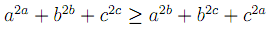Author(s):

A. Coronel and F. Huancas

Departamento de Ciencias Básicas,
Campus Fernando May, Chillán, Chile.

acoronel@roble.fdo-may.ubiobio.cl

Facultad de Ciencias Físicas y Matemáticas,
Universidad Nacional Pedro Ruiz Gallo, Juan XIII s/n,
Lambayeque, Perú

Abstract:

In this paper we give a complete proof offor all positive real numbers a, b and c. Furthermore, we present another way to prove the statement for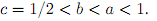1: Paper Source PDF document

Paper's Title:

Ellipses Inscribed in Parallelograms

Author(s):

A. Horwitz

Penn State University,
25 Yearsley Mill Rd.
Media, PA 19063
U. S. A.
alh4@psu.edu

Abstract:

We prove that there exists a unique ellipse of minimal eccentricity, EI, inscribed in a parallelogram, Đ. We also prove that the smallest nonnegative angle between equal conjugate diameters of \$EI equals the smallest nonnegative angle between the diagonals of Đ. We also prove that if EM is the unique ellipse inscribed in a rectangle, R, which is tangent at the midpoints of the sides of R, then EM is the unique ellipse of minimal eccentricity, maximal area, and maximal arc length inscribed in R. Let Đ be any convex quadrilateral. In previous papers, the author proved that there is a unique ellipse of minimal eccentricity, EI, inscribed in Đ, and a unique ellipse, EO, of minimal eccentricity circumscribed about Đ. We defined Đ to be bielliptic if EI and EO have the same eccentricity. In this paper we show that a parallelogram, Đ, is bielliptic if and only if the square of the length of one of the diagonals of Đ equals twice the square of the length of one of the sides of Đ .

1: Paper Source PDF document

Paper's Title:

Growth and Products of Subharmonic Functions in the Unit Ball

Author(s):

R. Supper

Université de Strasbourg,
UFR de Mathématique et Informatique, URA CNRS 001,
7 rue René Descartes,
F--67 084 Strasbourg Cedex,
France
raphaele.supper@math.unistra.fr

Abstract:

The purpose of this paper is to link information on the application u→gu with some growth conditions on the functions u and g subharmonic in the unit ball of RN. Two kinds of growth are considered: the Bloch--type growth and growth conditions expressed through integrals involving involutions of the unit ball.

1: Paper Source PDF document

Paper's Title:

Fejér-type Inequalities

Author(s):

Nicuşor Minculete and Flavia-Corina Mitroi

"Dimitrie Cantemir" University,
107 Bisericii Române Street, Braşov, 500068,
România
minculeten@yahoo.com

University of Craiova, Department of Mathematics,
Street A. I. Cuza 13, Craiova, RO-200585,
Romania
fcmitroi@yahoo.com

Abstract:

The aim of this paper is to present some new Fejér-type results for convex functions. Improvements of Young's inequality (the arithmetic-geometric mean inequality) and other applications to special means are pointed as well.

1: Paper Source PDF document

Paper's Title:

Existence Results for Second Order Impulsive Functional Differential Equations with Infinite Delay

Author(s):

M. Lakrib, A. Oumansour and K. Yadi

Laboratoire de Mathématiques, Université Djillali
Liabées, B.P. 89 Sidi Bel Abbčs 22000, Algérie
mlakrib@univ-sba.dz
oumansour@univ-sba.dz

Laboratoire de Mathématiques, Université Abou Bekr
Belkaid, B.P. 119 Tlemcen 13000, Algérie

Abstract:

In this paper we study the existence of solutions for second order impulsive functional differential equations with infinite delay. To obtain our results, we apply fixed point methods.

1: Paper Source PDF document

Paper's Title:

On the Boundedness of Hardy's Averaging Operators

Author(s):

Dah-Chin Luor

Department of Applied Mathematics,
I-Shou University, Dashu District,
Kaohsiung City 84001,
Taiwan, R.O.C.
dclour@isu.edu.tw

Abstract:

In this paper we establish scales of sufficient conditions for the boundedness of Hardy's averaging operators on weighted Lebesgue spaces. The estimations of the operator norms are also obtained. Included in particular are the Erdélyi-Kober operators.

1: Paper Source PDF document

Paper's Title:

Positive Solutions to a System of Boundary Value Problems for Higher-Dimensional Dynamic Equations on Time Scales

Author(s):

I. Y. Karaca

Department of Mathematics,
Ege University,
35100 Bornova, Izmir,
Turkey

ilkay.karaca@ege.edu.tr

URL: http://ege.edu.tr

Abstract:

In this paper, we consider the system of boundary value problems for higher-dimensional dynamic equations on time scales. We establish criteria for the existence of at least one or two positive solutions. We shall also obtain criteria which lead to nonexistence of positive solutions. Examples applying our results are also given.

1: Paper Source PDF document

Paper's Title:

Expected Utility with Subjective Events

Author(s):

Jacob Gyntelberg and Frank Hansen

Bank for International Settlements,
Basel,
Switzerland

jacob.gyntelberg@bis.org

Tohoku University, Institute for International Education,
Sendai,
Japan

frank.hansen@m.tohoku.ac.jp

Abstract:

We provide a new theory of expected utility with subjective events modeled by a lattice of projections. This approach allows us to capture the notion of a ``small world'' as a context dependent or local state space embedded into a subjective set of events, the ``grand world''. For each situation the decision makers' subjective ``small world'' reflects the events perceived to be relevant for the act under consideration. The subjective set of events need not be representable by a classical state space. Maintaining preference axioms similar in spirit to the classical axioms, we obtain an expected utility representation which is consistent across local state spaces and separates subjective probability and utility. An added benefit is that this alternative expected utility representation allows for an intuitive distinction between risk and uncertainty.

1: Paper Source PDF document

Paper's Title:

Properties of Certain Multivalent Functions Involving Ruscheweyh Derivatives

Author(s):

N-Eng Xu and Ding-Gong Yang

Department of Mathematics,
Changshu Institute of Technology,
Changshu, Jiangsu 215500,
China

Abstract:

Let Ap(p∈ N) be the class of functions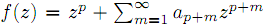which are analytic in the unit disk. By virtue of the Ruscheweyh derivatives we introduce the new subclasses Cp(n,α,β,λ,μ) of Ap. Subordination relations, inclusion relations, convolution properties and a sharp coefficient estimate are obtained. We also give a sufficient condition for a function to be in Cp(n,α,β,λ,μ)

1: Paper Source PDF document

Paper's Title:

On Reformations of 2--Hilbert Spaces

Author(s):

M. Eshaghi Gordji, A. Divandari, M. R. Safi and Y. J. Cho

Department of Mathematics, Semnan University,
P.O. Box 35195--363, Semnan,
Iran

Department of Mathematics, Semnan University,
Iran

Divandari@sun.Semnan.ac.ir

Department of Mathematics, Semnan University,
Iran

Department of Mathematics Education and the RINS,
Gyeongsang National University
Chinju 660-701,
Korea

yjcho@gnu.ac.kr

Abstract:

In this paper, first, we introduce the new concept of (complex) 2--Hilbert spaces, that is, we define the concept of 2--inner product spaces with a complex valued 2--inner product by using the 2--norm. Next, we prove some theorems on Schwartz's inequality, the polarization identity, the parallelogram laws and related important properties. Finally, we give some open problems related to 2--Hilbert spaces.

1: Paper Source PDF document

Paper's Title:

Some Operator Order Inequalities for Continuous Functions of Selfadjoint Operators in Hilbert Spaces

Author(s):

S. S. Dragomir1,2 and Charles E. M. Pearce3

1Mathematics, School of Engineering & Science,
Victoria University,
PO Box 14428, Melbourne City, MC 8001,
Australia.

2School of Computational & Applied Mathematics,
University of the Witwatersrand,
Private Bag 3, Johannesburg 2050,
South Africa.

sever.dragomir@vu.edu.au
URL: http://rgmia.org/dragomir

3School of Mathematical Sciences,
Australia

Abstract:

Various bounds in the operator order for the following operator transform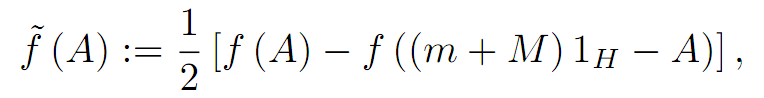where A is a selfadjoint operator in the Hilbert space H with the spectrum
Sp( A) ⊆ [ m,M] and f:[m,M] ->  C is a continuous function on [m,M]  are given. Applications for the power and logarithmic functions are provided as well.

1: Paper Source PDF document

Paper's Title:

A Dynamic Contact Problem for an Electro Viscoelastic Body

Author(s):

Denche M. and Ait Kaki L.

Laboratoire Equations Differentielles,
Departement de Mathematiques,
Universite Constantine 1,
Algeria.

Ecole Normale Superieure,
Departement des Sciences Exactes et Informatique,
Plateau Mansourah, Constantine.
Algeria.

Abstract:

We consider a dynamic problem which describes a contact between a piezoelectric body and a conductive foundation. The frictionless contact is modelled with the normal compliance, the electric conditions are supposed almost perfect. We prove the existence of a unique weak solution for almost perfect electric contact.

1: Paper Source PDF document

Paper's Title:

L∞- Error Estimate of Schwarz Algorithm for Elliptic Quasi-Variational Inequalities Related to Impulse Control Problem

Author(s):

Lab. LANOS, Department of Mathematics,
P.O.Box 12, Annaba 23000,
Algeria.

Lab. LAIG, Department of Mathematics,
University May 8th 1945,
P.O.Box 401, Guelma 24000,
Algeria.

allmehri@yahoo.fr

Abstract:

In this work, we study Schwarz method for a class of elliptic quasi-variational inequalities. The principal result of this investigation is to prove the error estimate in ∞-norm for two domains with overlapping nonmatching grids, using the geometrical convergence, and the uniform convergence of Cortey Dumont.

1: Paper Source PDF document

Paper's Title:

Scope of the Logarithmic Mean

Author(s):

Murali Rao and Agnish Dey

Department of Mathematics,
University of Florida,
Florida 32611,
U. S. A.

E-mail: mrao@ufl.edu

URL: http://people.clas.ufl.edu/mrao

E-mail: agnish@ufl.edu

URL: http://people.clas.ufl.edu/agnish

Abstract:

A number a is between two numbers x and y if and only if a is a convex combination of x and y, in other words, it is a "weighted mean" of x and y. Geometric mean, arithmetic mean are well known examples of these "means". Of more recent vintage is the logarithmic mean which has been considered in many articles in the literature. In this note, we first discuss some of its properties. Then we shall introduce the L function and explore the inverse of this function and its connection with the Lambert's Omega function.

1: Paper Source PDF document

Paper's Title:

Existence and Regularity of Minima of an Integral Functional in Unbounded Domain

Author(s):

L. Aharouch, J. Bennouna and A. Bouajaja

King Khalid University
Faculty of Arts and Science Mha'l Asir
Saudi Arabia.

E-mail: laharouch@gmail.com

Université Sidi Mohammed Ben Abdellah
Faculté des Sciences Dhar-Mahraz
B.P 1796 Atlas Fčs,
Maroc.

Email: jbennouna@hotmail.com

Abstract:

We prove the existence and the regularity of minima for a functional defined on a suitable Sobolev space.

1: Paper Source PDF document

Paper's Title:

Examples of Fractals Satisfying the Quasihyperbolic Boundary Condition

Author(s):

Petteri Harjulehto and Riku Klén

Department of Mathematics and Statistics,
FI-20014 University of Turku,
Finland

E-mail: petteri.harjulehto@utu.fi

E-mail: riku.klen@utu.fi

Abstract:

In this paper we give explicit examples of bounded domains that satisfy the quasihyperbolic boundary condition and calculate the values for the constants. These domains are also John domains and we calculate John constants as well. The authors do not know any other paper where exact values of parameters has been estimated.

1: Paper Source PDF document

Paper's Title:

Commutators of Hardy Type Operators

Author(s):

Chunping Xie

Department of Mathematics,
Milwaukee School of Engineering,
Milwaukee, Wisconsin 53202,
USA.

E-mail: xie@msoe.edu
URL: http://www.msoe.edu/people/chunping.xie

Abstract:

The note deals with commutaors of the Hardy operator, Hardy type operators on Morrey spaces on R+. We have proved that the commutators generated by Hardy operator and Hardy type operators with a BMO function b are bounded on the Morrey spaces.

1: Paper Source PDF document

Paper's Title:

On the Hyers-Ulam Stability of Homomorphisms and Lie Derivations

Author(s):

Department of Mathematics, Payame Noor University,
P.O. Box: 19395-3697, Tehran,
Iran.

Abstract:

Let A be a Lie Banach*-algebra. For each elements (a, b) and (c, d) in A2:= A * A, by definitions

(a, b) (c, d)= (ac, bd),
|(a, b)|= |a|+ |b|,
(a, b)*= (a*, b*),

A2 can be considered as a Banach*-algebra. This Banach*-algebra is called a Lie Banach*-algebra whenever it is equipped with the following definitions of Lie product: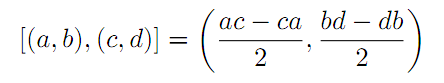for all a, b, c, d in A. Also, if A is a Lie Banach*-algebra, then D: A2→A2 satisfying

D ([ (a, b), (c, d)])= [ D (a, b), (c, d)]+ [(a, b), D (c, d)]

for all \$a, b, c, d∈A, is a Lie derivation on A2. Furthermore, if A is a Lie Banach*-algebra, then D is called a Lie* derivation on A2 whenever D is a Lie derivation with D (a, b)*= D (a*, b*) for all a, b∈A. In this paper, we investigate the Hyers-Ulam stability of Lie Banach*-algebra homomorphisms and Lie* derivations on the Banach*-algebra A2.

1: Paper Source PDF document

Paper's Title:

Some New Generalizations of Jensen's Inequality with Related Results and Applications

Author(s):

Steven G. From

Department of Mathematics

E-mail: sfrom@unomaha.edu

Abstract:

In this paper, some new generalizations of Jensen's inequality are presented. In particular, upper and lower bounds for the Jensen gap are given and compared analytically and numerically to previously published bounds for both the discrete and continuous Jensen's inequality cases. The new bounds compare favorably to previously proposed bounds. A new method based on a series of locally linear interpolations is given and is the basis for most of the bounds given in this paper. The wide applicability of this method will be demonstrated. As by-products of this method, we shall obtain some new Hermite-Hadamard inequalities for functions which are 3-convex or 3-concave. The new method works to obtain bounds for the Jensen gap for non-convex functions as well, provided one or two derivatives of the nonlinear function are continuous. The mean residual life function of applied probability and reliability theory plays a prominent role in construction of bounds for the Jensen gap. We also present an exact integral representation for the Jensen gap in the continuous case. We briefly discuss some inequalities for other types of convexity, such as convexity in the geometric mean, and briefly discuss applications to reliability theory.

1: Paper Source PDF document

Paper's Title:

Hermite-Hadamard Type Inequalities for k-Riemann Liouville Fractional Integrals Via Two Kinds of Convexity

Author(s):

R. Hussain1, A. Ali2, G. Gulshan3, A. Latif4 and K. Rauf5

1,2,3,4Department of Mathematics,
Mirpur University of Science and Technology, Mirpur.
Pakistan.
E-mail1rashida12@gmail.com
E-mail2: unigraz2009@yahoo.com
E-mail3: ghazalagulshan@yahoo.com
E-mail4: asialatif87@gmail.com

5Department of Mathematics,
University of Ilorin, Ilorin,
Nigeria.
E-mail5: krauf@unilorin.edu.ng

Abstract:

In this article, a fundamental integral identity including the first order derivative of a given function via k-Riemann-Liouville fractional integral is established. This is used to obtain further Hermite-Hadamard type inequalities involving left-sided and right-sided k-Riemann-Liouville fractional integrals for m-convex and (s,m)-convex functions respectively.

1: Paper Source PDF document

Paper's Title:

On The Rayleigh-Love Rod Accreting In Both Length And Cross-Sectional Area: Forced And Damped Vibrations

Author(s):

M.L.G. Lekalakala1, M. Shatalov2, I. Fedotov3, S.V. Joubert4

1Department of Mathematics, Vaal University of Technology, P.O. Box 1889, Secunda, 2302, South Africa.
E-mail1: glen@vut.ac.za

2,3,4Department of Mathematics and Statistics, Tshwane University of Technology, Pretoria, South Africa.

Abstract:

In this paper an elastic cylindrical rod that is subjected to forced and damped vibrations is considered. The rod is assumed to be isotropic. The applied external force of excitation is assumed to be harmonic, and the damping force is that of Kelvin-Voigt. The longitudinally vibrating rod is fixed at the left end and free at the other end. The rod is assumed to be accreting in length and cross-sectional area as it vibrates. The problem arising and the dynamics of the vibrating rod are described and investigated within the Rayleigh-Love theories of the rod. A partial differential equation describing the longitudinal displacement of the rod is formulated. The formulated partial differential equation, together with the corresponding boundary conditions as per the configuration of the rod, is solved numerically using the Galerkin-Kantorovich method. The frequency of vibration of the harmonic exciting force is kept constant in this investigation.

It is shown that in this periodically forced viscoelastic damped vibration, all the modes of vibration are subjected to the resonance behaviour within a proper time interval, depending on the length of the accreting rod.

1: Paper Source PDF document

Paper's Title:

Bilinear Regular Operators on Quasi-Normed Functional Spaces

Author(s):

D. L. Fernandez and E. B. Silva

Instituto de Matematica
Campinas - SP
Brazil.
E-mail: dicesar@ime.unicamp.br

Departamento de Matematica
Av.~Colombo 5790
Maringa - PR
Brazil.
E-mail: ebsilva@uem.br

Abstract:

Positive and regular bilinear operators on quasi-normed functional spaces are introduced and theorems characterizing compactness of these operators are proved. Relations between bilinear operators and their adjoints in normed functional spaces are also studied.

1: Paper Source PDF document

Paper's Title:

Wavelet Frames in Higher Dimensional Sobolev Spaces

Author(s):

Raj Kumar, Manish Chauhan, and Reena

Department of Mathematics,
Kirori Mal College, University of Delhi,
New Delhi-110007,
India.
E-mail: rajkmc@gmail.com

Department of Mathematics,
University of Delhi,
New Delhi-110007,
India
E-mail: manish17102021@gmail.com

Department of Mathematics,
Hans Raj College, University of Delhi,
New Delhi-110007,
India
E-mail: reena.bhagwat29@gmail.com

Abstract:

In this paper, we present sufficient condition for the sequence of vectors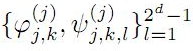to be a frame for Hs(Rd) are derived. Necessary and sufficient conditions for the sequence of vectorsto be tight wavelet frames in Hs(Rd) are obtained. Further, as an application an example of tight wavelet frames for Hs(R2) as bivariate box spline over 3-direction are given.

1: Paper Source PDF document

Paper's Title:

A Note on Calderon Operator

Author(s):

Chunping Xie

Department of Mathematics,
Milwaukee School of Engineering,
Milwaukee, Wisconsin 53202,
U. S. A
.

E-mail: xie@msoe.edu

URL: http://www.msoe.edu/people/chunping.xie

Abstract:

We have shown that the Calderon operator is bounded on Morrey Spaces on R+. Also under certain conditions on the weight, the Hardy operator, the adjoint Hardy operator, and therefore the Caldern operator are bounded on the weighted Morrey spaces.

1: Paper Source PDF document

Paper's Title:

Inequalities for Discrete F-Divergence Measures: A Survey of Recent Results

Author(s):

Sever S. Dragomir1,2

1Mathematics, School of Engineering & Science
Victoria University, PO Box 14428
Melbourne City, MC 8001,
Australia
E-mail: sever.dragomir@vu.edu.au

2DST-NRF Centre of Excellence in the Mathematical and Statistical Sciences,
School of Computer Science & Applied Mathematics,
University of the Witwatersrand,
Private Bag 3, Johannesburg 2050,
South Africa
URL: http://rgmia.org/dragomir

Abstract:

In this paper we survey some recent results obtained by the author in providing various bounds for the celebrated f-divergence measure for various classes of functions f. Several techniques including inequalities of Jensen and Slater types for convex functions are employed. Bounds in terms of Kullback-Leibler Distance, Hellinger Discrimination and Varation distance are provided. Approximations of the f-divergence measure by the use of the celebrated Ostrowski and Trapezoid inequalities are obtained. More accurate approximation formulae that make use of Taylor's expansion with integral remainder are also surveyed. A comprehensive list of recent papers by several authors related this important concept in information theory is also included as an appendix to the main text.

1: Paper Source PDF document

Paper's Title:

Generalized k-distance-balanced Graphs

Author(s):

Amir Hosseini and Mehdi Alaeiyan

Department of mathematics, Karaj Branch,
Iran.
E-mail: amir.hosseini@kiau.ac.ir, hosseini.sam.52@gmail.com

Department of Mathematics,
Iran University of Science and Technology, Tehran,
Iran.
E-mail: alaeiyan@iust.ac.ir

Abstract:

A nonempty graph Γ is called generalized k-distance-balanced, whenever every edge ab has the following property: the number of vertices closer to a than to b, k, times of vertices closer to b than to a, or conversely, k N .In this paper we determine some families of graphs that have this property, as well as to prove some other result regarding these graphs.

1: Paper Source PDF document

Paper's Title:

A Multivalued Version of the Radon-Nikodym Theorem, via the Single-valued Gould Integral

Author(s):

Domenico Candeloro1, Anca Croitoru2, Alina Gavriluţ2, Anna Rita Sambucini1

1Dept. of Mathematics and Computer Sciences,
University of Perugia,
1, Via Vanvitelli -- 06123, Perugia,
Italy.
E-mail:  domenico.candeloro@unipg.it, anna.sambucini@unipg.it

2Faculty of Mathematics,
Al. I. Cuza University,
700506 Iaşi,
Romania.
E-mail: croitoru@uaic.ro, gavrilut@uaic.ro

Abstract:

In this paper we consider a Gould type integral of real functions with respect to a compact and convex valued not necessarily additive measure. In particular we will introduce the concept of integrable multimeasure and, thanks to this notion, we will establish an exact Radon-Nikodym theorem relative to a fuzzy multisubmeasure which is new also in the finite dimensional case. Some results concerning the Gould integral are also obtained.

1: Paper Source PDF document

Paper's Title:

Iterative Algorithm for Split Generalized Mixed Equilibrium Problem Involving Relaxed Monotone Mappings in Real Hilbert Spaces

Author(s):

1U.A. Osisiogu, F.L. Adum, and 2C. Izuchukwu

1Department of Mathematics and Computer Science,
Ebonyi State University, Abakaliki,
Nigeria.

2School of Mathematics, Statistics and Computer Science,
University of KwaZulu-Natal, Durban,
South Africa.
E-mail: izuchukwuc@ukzn.ac.za, izuchukwu_c@yahoo.com

Abstract:

The main purpose of this paper is to introduce a certain class of split generalized mixed equilibrium problem involving relaxed monotone mappings. To solve our proposed problem, we introduce an iterative algorithm and obtain its strong convergence to a solution of the split generalized mixed equilibrium problems in Hilbert spaces. As special cases of the proposed problem, we studied the proximal split feasibility problem and variational inclusion problem.

1: Paper Source PDF document

Paper's Title:

A Note on Taylor Expansions Without the Differentiability Assumption

Author(s):

Moawia Alghalith

Economics Dept.,
University of the West Indies,
St Augustine,

E-mail: malghalith@gmail.com

Abstract:

We introduce new Taylor expansions when the function is not differentiable.

Retraction

This article has been retracted under the authority of the Editor on Chief on 1 April, 2021. The PDF file is no longer available, but can be obtained from the editorial office upon request.

1: Paper Source PDF document

Paper's Title:

Some Inequalities of the Hermite-Hadamard Type for k-Fractional Conformable Integrals

Author(s):

C.-J. Huang, G. Rahman, K. S. Nisar, A. Ghaffar and F. Qi

Department of Mathematics, Ganzhou Teachers College,
Ganzhou 341000, Jiangxi,
China.
E-mail: hcj73jx@126.com , huangcj1973@qq.com

Department of Mathematics, Shaheed Benazir Bhutto University,
Sheringal, Upper Dir, Khyber Pakhtoonkhwa,
Pakistan.
E-mail: gauhar55uom@gmail.com

Department of Mathematics, College of Arts and Science at Wadi Aldawaser, 11991,
Prince Sattam Bin Abdulaziz University, Riyadh Region,
Kingdom of Saudi Arabia.
E-mail: n.sooppy@psau.edu.sa, ksnisar1@gmail.com

Department of Mathematical Science, Balochistan University of Information Technology,
Engineering and Management Sciences, Quetta,
Pakistan.
E-mail: abdulghaffar.jaffar@gmail.com

School of Mathematical Sciences, Tianjin Polytechnic University,
Tianjin 300387,
China; Institute of Mathematics,
Henan Polytechnic University, Jiaozuo 454010, Henan,
China.
E-mail: qifeng618@gmail.com, qifeng618@qq.com

Abstract:

In the paper, the authors deal with generalized k-fractional conformable integrals, establish some inequalities of the Hermite-Hadamard type for generalized k-fractional conformable integrals for convex functions, and generalize known inequalities of the Hermite-Hadamard type for conformable fractional integrals.

1: Paper Source PDF document

Paper's Title:

On a New Class of Eulerian's Type Integrals Involving Generalized Hypergeometric Functions

Author(s):

Sungtae Jun, Insuk Kim and Arjun K. Rathie

General Education Institute,
Konkuk University, Chungju 380-701,
Republic of Korea.

Department of Mathematics Education,
Wonkwang University, Iksan, 570-749,
Republic of Korea.

Department of Mathematics,
Vedant College of Engineering and Technology (Rajasthan Technical University),
Bundi-323021, Rajasthan,
India.

E-mail: sjun@kku.ac.kr, iki@wku.ac.kr, arjunkumarrathie@gmail.com

Abstract:

Very recently Masjed-Jamei and Koepf established interesting and useful generalizations of various classical summation theorems for the 2F1, 3F2, 4F3, 5F4 and 6F5 generalized hypergeometric series. The main aim of this paper is to establish eleven Eulerian's type integrals involving generalized hypergeometric functions by employing these theorems. Several special cases have also been given.

1: Paper Source PDF document

Paper's Title:

Some Convergence Results for  Jungck-Am Iterative Process In Hyperbolic Spaces

Author(s):

Akindele Adebayo Mebawondu and Oluwatosin Temitope Mewomo

School of Mathematics, Statistics and Computer Science,
University of KwaZulu-Natal, Durban,
South Africa.
E-mail: 216028272@stu.ukzn.ac.za, mewomoo@ukzn.ac.za

Abstract:

In this paper, we introduce a new three steps iterative process called Jungck-AM iterative process and show that the proposed iterative process can be used to approximate fixed points of Jungck-contractive type mappings and Jungck-Suzuki type mappings. In addition, we establish some strong and Δ-convergence results for the approximation of fixed points of Jungck-Suzuki type mappings in the frame work of uniformly convex hyperbolic space. Furthermore, we show that the newly proposed iterative process has a better rate of convergence compare to the Jungck-Noor, Jungck-SP, Jungck-CR and some existing iterative processes in the literature. Finally, stability, data dependency results for Jungck-AM iterative process is established and we present an analytical proof and numerical examples to validate our claim.

1: Paper Source PDF document

Paper's Title:

Dynamical Analysis of HIV/AIDS Epidemic Model with Two Latent Stages, Vertical Transmission and Treatment

Author(s):

Nur Shofianah, Isnani Darti, Syaiful Anam

Mathematics Department,Faculty of Mathematics and Natural Sciences.
University of Brawijaya,
Jl. Veteran, Malang 65145,
Indonesia.
E-mail: nur_shofianah@ub.ac.id, isnanidarti@ub.ac.id, syaiful@ub.ac.id

Abstract:

We discuss about dynamical analysis of HIV/AIDS epidemic model with two latent stages, vertical transmission and treatment. In this model, the spreading of HIV occurs through both horizontal and vertical transmission. There is also treatment for individual who has been HIV infected. The latent stage is divided into slow and fast latent stage based on the immune condition which varies for each individual. Dynamical analysis result shows that the model has two equilibrium points: the disease-free equilibrium point and the endemic equilibrium point. The existence and global stability of equilibrium points depend on the basic reproduction number R0. When R0 <1, only the disease-free equilibrium point exists. If R0 >1, there are two equilibrium points, which are the disease-free equilibrium point and the endemic equilibrium point. Based on the result of stability analysis, the disease-free equilibrium point is globally asymptotically stable if R0 <1, while if R0 > 1 and p=q, the endemic equilibrium point will be globally asymptotically stable. In the end, we show some numerical simulations to support the analytical result.

1: Paper Source PDF document

Paper's Title:

A Low Order Least-Squares Nonconforming Finite Element Method for Steady Magnetohydrodynamic Equations

Author(s):

Z. Yu, D. Shi and H. Zhu

College of Science,
Zhongyuan University of Technology,
Zhengzhou 450007,
China.
E-mail: 5772@zut.edu.cn

School of Mathematics and Statistics,
Zhengzhou University,
Zhengzhou 450001,
China.
E-mail: shi_dy@126.com

Mathematics Department,
University of Southern Mississippi,
Hattiesburg MS, 39406,
U.S.A
E-mail: huiqing.zhu@usm.edu

Abstract:

A low order least-squares nonconforming finite element (NFE) method is proposed for magnetohydrodynamic equations with EQ1rot element and zero-order Raviart-Thomas element. Based on the above element's typical interpolations properties, the existence and uniqueness of the approximate solutions are proved and the optimal order error estimates for the corresponding variables are derived.

1: Paper Source PDF document

Paper's Title:

Kinematic Model for Magnetic Null-points in 2 Dimensions

Author(s):

Ali Khalaf Hussain Al-Hachami

Department of Mathematics,
College of Education For Pure Sciences,
Wasit University,
Iraq.
E-mail: alhachamia@uowasit.edu.iq

Abstract:

The adjacent configurations of two-dimensional magnetic null point centers are analyzed by an immediate examination about the null. The configurations are classified as either potential or non-potential. By then the non-potential cases are subdivided into three cases depending upon whether the component of current is less than, equal to or greater than a threshold current. In addition the essential structure of reconnection in 2D is examined. It unfolds that the manner by which the magnetic flux is rebuilt. In this paper, we center on the ramifications of kinematic arrangements; that is, we fathom just Maxwell's conditions and a resistive Ohm's law.

1: Paper Source PDF document

Paper's Title:

Global Analysis on Riemannian Manifolds

Author(s):

Louis Omenyi and Michael Uchenna

Department of Mathematics, Computer Science, Statistics and Informatics,
Alex Ekwueme Federal University, Ndufu-Alike,
Nigeria.
E-mail: omenyi.louis@funai.edu.ng, michael.uchenna@funai.edu.ng
URL: http://www.funai.edu.ng

Abstract:

In this paper, an exposition of the central concept of global analysis on a Riemannan manifold is given. We extend the theory of smooth vector fields from open subsets of Euclidean space to Riemannan manifolds. Specifically, we prove that a Riemannian manifold admits a unique solution for a system of ordinary differential equations generated by the flow of smooth tangent vectors. The idea of partial differential equations on Riemannian manifold is highlighted on the unit sphere.

1: Paper Source PDF document

Paper's Title:

Analysis of a Frictional Contact Problem for Viscoelastic Piezoelectric Materials

Author(s):

Meziane Said Ameur, Tedjani Hadj Ammar and Laid Maiza

Departement of Mathematics,
El Oued University,
P.O. Box 789, 39000 El Oued,
Algeria.
E-mail: said-ameur-meziane@univ-eloued.dz

Departement of Mathematics,
El Oued University,
P.O. Box 789, 39000 El Oued,
Algeria.

Department of Mathematics,
Kasdi Merbah University,
30000 Ouargla,
Algeria.
E-mail: maiza.laid@univ-ouargla.dz

Abstract:

In this paper, we consider a mathematical model that describes the quasi-static process of contact between two thermo-electro-viscoelastic bodies with damage and adhesion. The damage of the materials caused by elastic deformations. The contact is frictional and modeled with a normal compliance condition involving adhesion effect of contact surfaces. Evolution of the bonding field is described by a first order differential equation. We derive variational formulation for the model and prove an existence and uniqueness result of the weak solution. The proof is based on arguments of evolutionary variational inequalities, parabolic inequalities, differential equations, and fixed point theorem.

1: Paper Source PDF document

Paper's Title:

Oblique Projectors from the Simpson Discrete Fourier Transformation Matrix

Author(s):

P. Singh and V. Singh

School of Mathematics, Computer Science and Statistics,
University of Kwazulu-Natal,
Private Bag X54001, Durban 4001,
South Africa.
E-mail: singhp@ukzn.ac.za, singhv@ukzn.ac.za

Abstract:

In this paper we examine the projectors of the Simpson Discrete Fourier Transform matrix of dimension two modulus four and show how they decompose the complex vector space into a direct sum of oblique eigenspaces. These projection operators are used to define a Simpson Discrete Fractional Fourier Transform (SDFRFT).

1: Paper Source PDF document

Paper's Title:

An Efficient Modification of Differential Transform Method for Solving Integral and Integro-differential Equations

Author(s):

S. Al-Ahmad, Ibrahim Mohammed Sulaiman*, and M. Mamat

Faculty of Informatics and Computing,
Universiti Sultan Zainal Abidin,
Terengganu, Besut Campus, 22200,
Malaysia.

Abstract:

In this paper, classes of integral and integro-differential equations are solved using a modified differential transform method. This proposed technique is based on differential transform method (DTM), Laplace transform (LT) procedure and Pad\'{e} approximants (PA). The proposed method which gives a good approximation for the true solution in a large region is referred to modified differential transform method (MDTM). An algorithm was developed to illustrate the flow of the proposed method. Some numerical problems are presented to check the applicability of the proposed scheme and the obtained results from the computations are compared with other existing methods to illustrates its efficiency. Numerical results have shown that the proposed MDTM method is promising compared to other existing methods for solving integral and integro-differential equations.

1: Paper Source PDF document

Paper's Title:

Analysis of a Dynamic Elasto-viscoplastic Frictionless Antiplan Contact Problem with Normal Compliance

Author(s):

A. Ourahmoun1, B. Bouderah2, T. Serrar3

1,2Applied Mathematics Laboratory,
M'sila University, 28000,
Algeria.
E-mail: ourahmounabbes@yahoo.fr

3Applied Mathematics Laboratory,
Setif 1 University, 19000,
Algeria.

Abstract:

We consider a mathematical model which describes the dynamic evolution of a thermo elasto viscoplastic contact problem between a body and a rigid foundation. The mechanical and thermal properties of the obstacle coating material near its surface. A variational formulation of this dynamic contact phenomenon is derived in the context of general models of thermo elasto viscoplastic materials. The displacements and temperatures of the bodies in contact are governed by the coupled system consisting of a variational inequality and a parabolic differential equation. The proof is based on a classical existence and uniqueness result on parabolic inequalities,differential equations and fixed point arguments.

1: Paper Source PDF document

Paper's Title:

Optimization Techniques on Affine Differential Manifolds

Author(s):

Ali S Rasheed, Faik Mayah and Ahmed A H AL-Jumaili

Ministry of Higher Education and Scientific Research,
Iraq.
E-mail: ahmedhashem@gmail.com

Department of Physics, College of Sciences,
University of Wasit,
Iraq.
E-mail: faik.mayah@gmail.com

Abstract:

In addition to solid ground of Riemannian manifolds fundamentals, this article interviews some popular optimization methods on Riemannian manifolds. Several optimization problems can be better stated on manifolds rather than Euclidean space, such as interior point methods, which in turns based on self-concordant functions (logarithmic barrier functions). Optimization schemes like the steepest descent scheme, the Newton scheme, and others can be extended to Riemannian manifolds. This paper introduces some Riemannian and non-Riemannian schemes on manifolds.

1: Paper Source PDF document

Paper's Title:

Sweeping Surfaces with Darboux Frame in Euclidean 3-space E3

Author(s):

F. Mofarreh, R. Abdel-Baky and N. Alluhaibi

Mathematical Science Department, Faculty of Science,
Princess Nourah bint Abdulrahman University
Saudi Arabia.
E-mail: fyalmofarrah@pnu.edu.sa

Department of Mathematics, Faculty of Science,
University of Assiut,
Assiut 71516,
Egypt.
E-mail: rbaky@live.com

Department of Mathematics Science and Arts, College Rabigh Campus,
King Abdulaziz University
Jeddah,
Saudi Arabia.
E-mail: nallehaibi@kau.edu.sa

Abstract:

The curve on a regular surface has a moving frame and it is called Darboux frame. We introduce sweeping surfaces along the curve relating to the this frame and investigate their geometrical properties. Moreover, we obtain the necessary and sufficient conditions for these surfaces to be developable ruled surfaces. Finally, an example to illustrate the application of the results is introduced.

1: Paper Source PDF document

Paper's Title:

Construction of a Frame Multiresolution Analysis on Locally Compact Abelian Groups

Author(s):

R. Kumar and Satyapriya

Department of Mathematics,
Kirori Mal College,
University of Delhi,
Delhi,
India.
E-mail: rajkmc@gmail.com

Department of Mathematics,
University of Delhi,
Delhi,
India.
E-mail: kmc.satyapriya@gmail.com

Abstract:

The frame multiresolution analysis (FMRA) on locally compact Abelian groups has been studied and the results concerning classical MRA have been worked upon to obtain new results. All the necessary conditions, which need to be imposed on the scaling function φ to construct a wavelet frame via FMRA, have been summed up. This process of construction of FMRA has aptly been illustrated by sufficient examples.

1: Paper Source PDF document

Paper's Title:

Analysis of the Dynamic Response of the Soil-pile Behavioral Model Under Lateral Load

Author(s):

Ibrahima Mbaye, Mamadou Diop, Aliou Sonko and Malick Ba

University of Thies,
Department of Mathematics, Bp 967 Thies,
Senegal.
E-mail: imbaye@univ-thies.sn
aliousonko59@gmail.com
mmalickba@hotmail.fr
URL: https://www.univ-thies.sn

Abstract:

This work aims to extend and improve our previous study on mathematical and numerical analysis of stationary Pasternak model. In this paper a dynamic response of Pasternak model is considered. On the one hand we establish the existence and uniqueness of the solution by using the Lax-Milgram theorem and the spectral theory thus the existence of a Hilbert basis is shown and the spectral decomposition of any solution of the problem can be established and on the other hand the finite element method is used to determinate the numerical results. Furthermore, the influence of soil parameters Gp and Kp on the displacement of the pious is studied numerically at any time tn.

1: Paper Source PDF document

Paper's Title:

Evaluation of a New Class of Double Integrals Involving Generalized Hypergeometric Function 4F3

Author(s):

Joohyung Kim, Insuk Kim and Harsh V. Harsh

Department of Mathematics Education,
Wonkwang University, Iksan, 570-749,
Korea.
E-mail: joohyung@wku.ac.kr

Department of Mathematics Education,
Wonkwang University, Iksan, 570-749,
Korea.
E-mail: iki@wku.ac.kr

Department of Mathematics, Amity School of Eng. and Tech.,
Amity University Rajasthan
NH-11C, Jaipur-303002, Rajasthan,
India.
E-mail: harshvardhanharsh@gmail.com

Abstract:

Very recently, Kim evaluated some double integrals involving a generalized hypergeometric function 3F2 with the help of generalization of Edwards's well-known double integral due to Kim,  et al. and generalized classical Watson's summation theorem obtained earlier by Lavoie,  et al. In this research paper we evaluate one hundred double integrals involving generalized hypergeometric function 4F3 in the form of four master formulas (25 each) viz. in the most general form for any integer. Some interesting results have also be obtained as special cases of our main findings.

1: Paper Source PDF document

Paper's Title:

Pointwise Convergence of Fourier-type Series with Exponential Weights

Author(s):

Hee Sun Jung and Ryozi Sakai

Department of Mathematics Education, Sungkyunkwan University,
Seoul 110-745,
Republic of Korea.
E-mail: hsun90@skku.edu

Department of Mathematics,
Meijo University, Nagoya 468-8502,
Japan.
E-mail: ryozi@hm.aitai.ne.jp

Abstract:

Let R = ( - ∞,∞), and let Q∈C1(R):R→[0,∞) be an even function. We consider the exponential weights w(x)=e-Q(x), xR. In this paper we obtain a pointwise convergence theorem for the Fourier-type series with respect to the orthonormal polynomials {pn(w2;x)}.

1: Paper Source PDF document

Paper's Title:

Semivectorial Bilevel Optimization on Affine-Finsler-Metric Manifolds

Author(s):

Faik Mayah1, Ali S Rasheed2 and Naseif J. Al- Jawari3

1Department of Physics,
College of Sciences,
University of Wasit,
Iraq.
E-mail: faik.mayah@gmail.com

2Ministry of Higher Education and Scientific Research,
Iraq.
E-mail: ali.math2018@yahoo.com ahmedhashem@gmail.com

3Dept. of Mathematics,
College of Science,
Iraq.
E-mail: nsaif642014@yahoo.com

Abstract:

A Finsler manifold is a differential manifold together with a Finsler metric, in this paper we construct a new class of Finsler metric affine manifolds on bilevel semivectorial with optimization problems. The first steps for this purpose involve the study of bilevel optimization on affine manifolds. The bilevel programming problem can be viewed as a static version of the noncooperative, two-person game which was introduced in the context of unbalanced economic markets. Bilevel optimization is a special kind of optimization where one problem is embedded within another.

1: Paper Source PDF document

Paper's Title:

On Euler's First Transformation Formula for k-hypergeometric Function

Author(s):

Sungtae Jun and Insuk Kim

General Education Institute,
Konkuk University, Chungju 380-701,
Republic of Korea.
E-mail: sjun@kku.ac.kr

Department of Mathematics Education,
Wonkwang University, Iksan, 570-749,
Republic of Korea.
E-mail: iki@wku.ac.kr

Abstract:

Mubeen et al. obtained Kummer's first transformation for the k-hypergeometric function. The aim of this note is to provide the Euler-type first transformation for the k-hypergeometric function. As a limiting case, we recover the results of Mubeen et al. In addition to this, an alternate and easy derivation of Kummer's first transformation for the k-hypergeometric function is also given.

Search and serve lasted 1 second(s).